# RD Sharma Solutions for Class 10 Maths Chapter 2 Polynomials

Mathematics is one of the scoring subjects in class 10. And that’s why we at BYJU’S have created the RD Sharma Solutions. This is created by our expert faculty in order to provide a clear understanding of important concepts by giving detailed explanations for the problems. This is a very useful tool for any student to get top marks in their Mathematics examinations.

## Download RD Sharma Solutions For Class 10 Maths Chapter 2 Polynomials PDF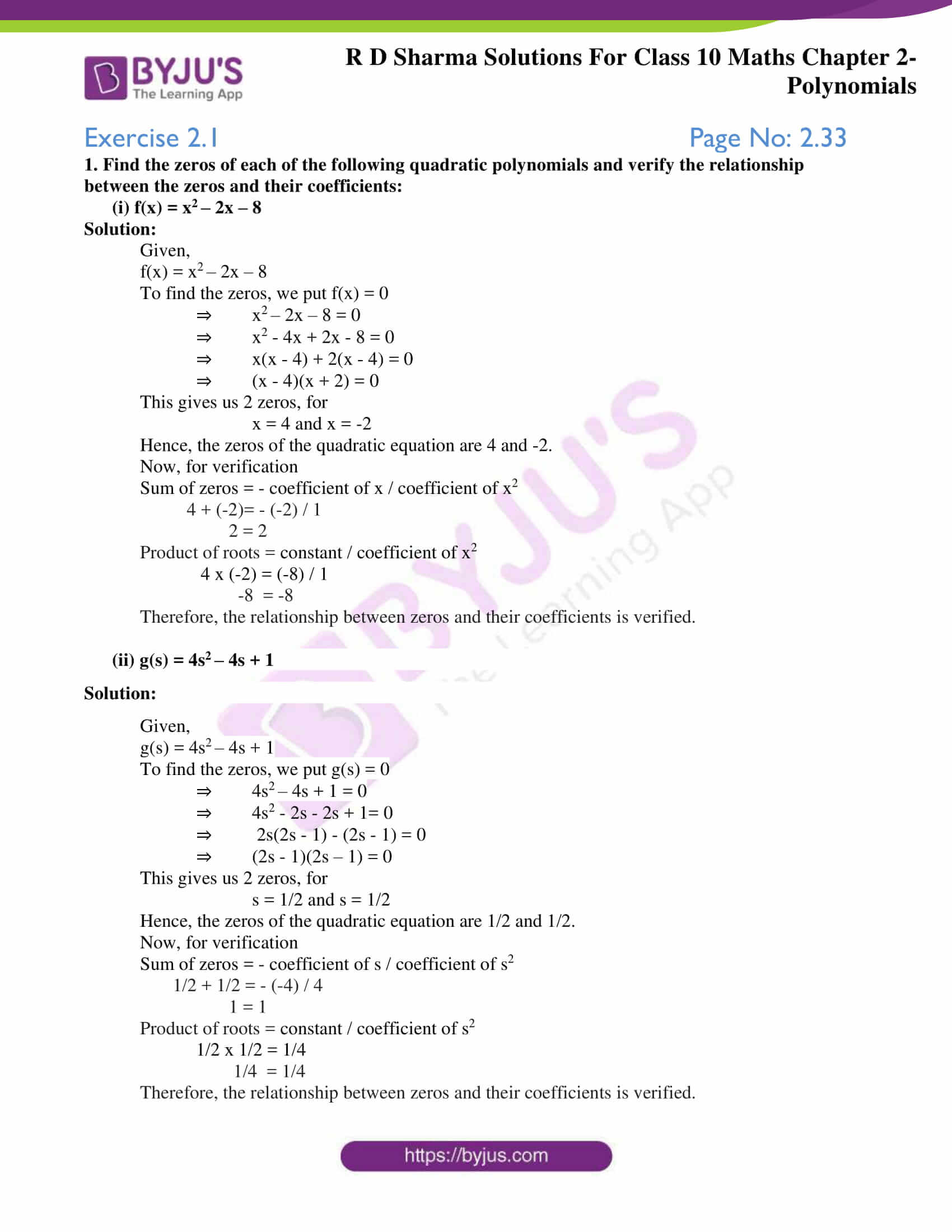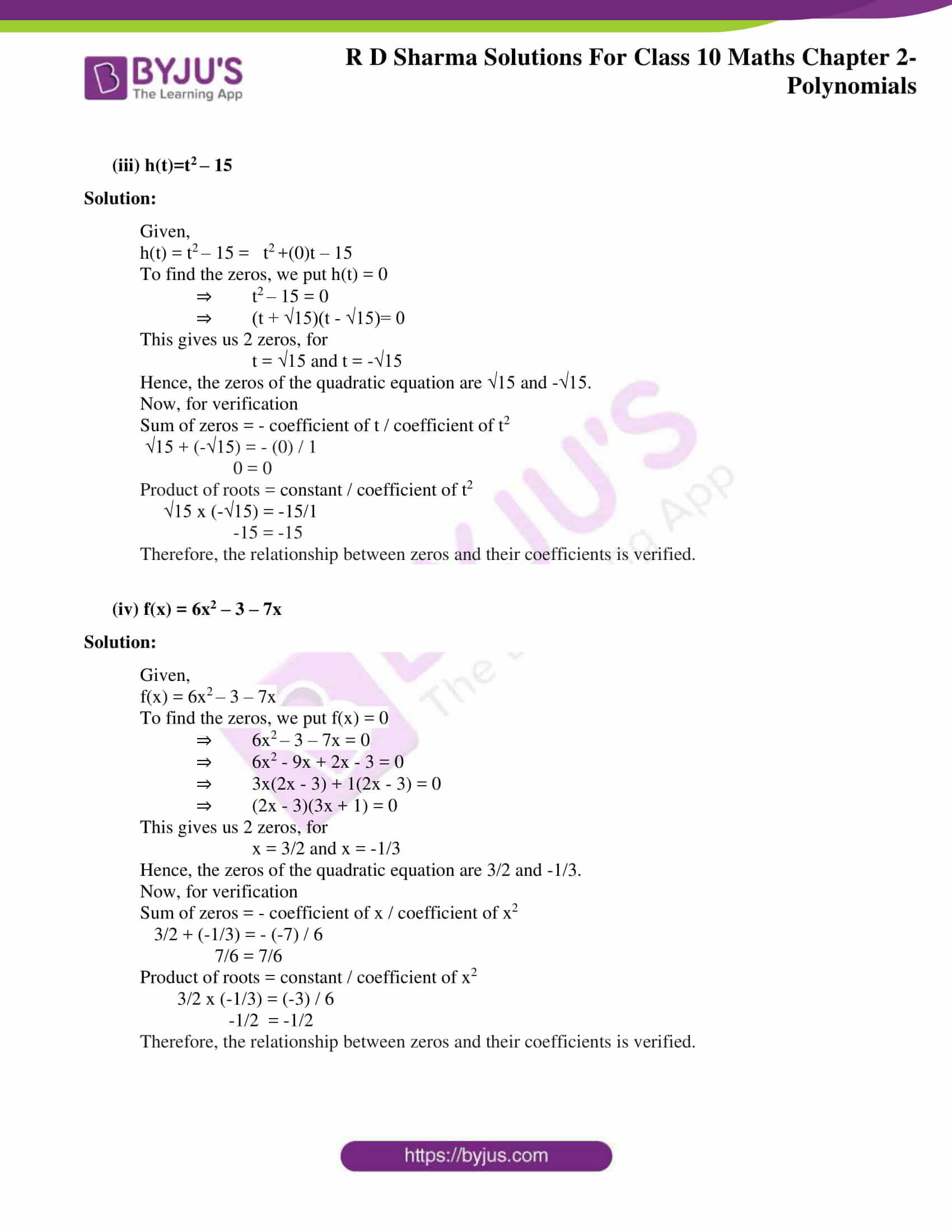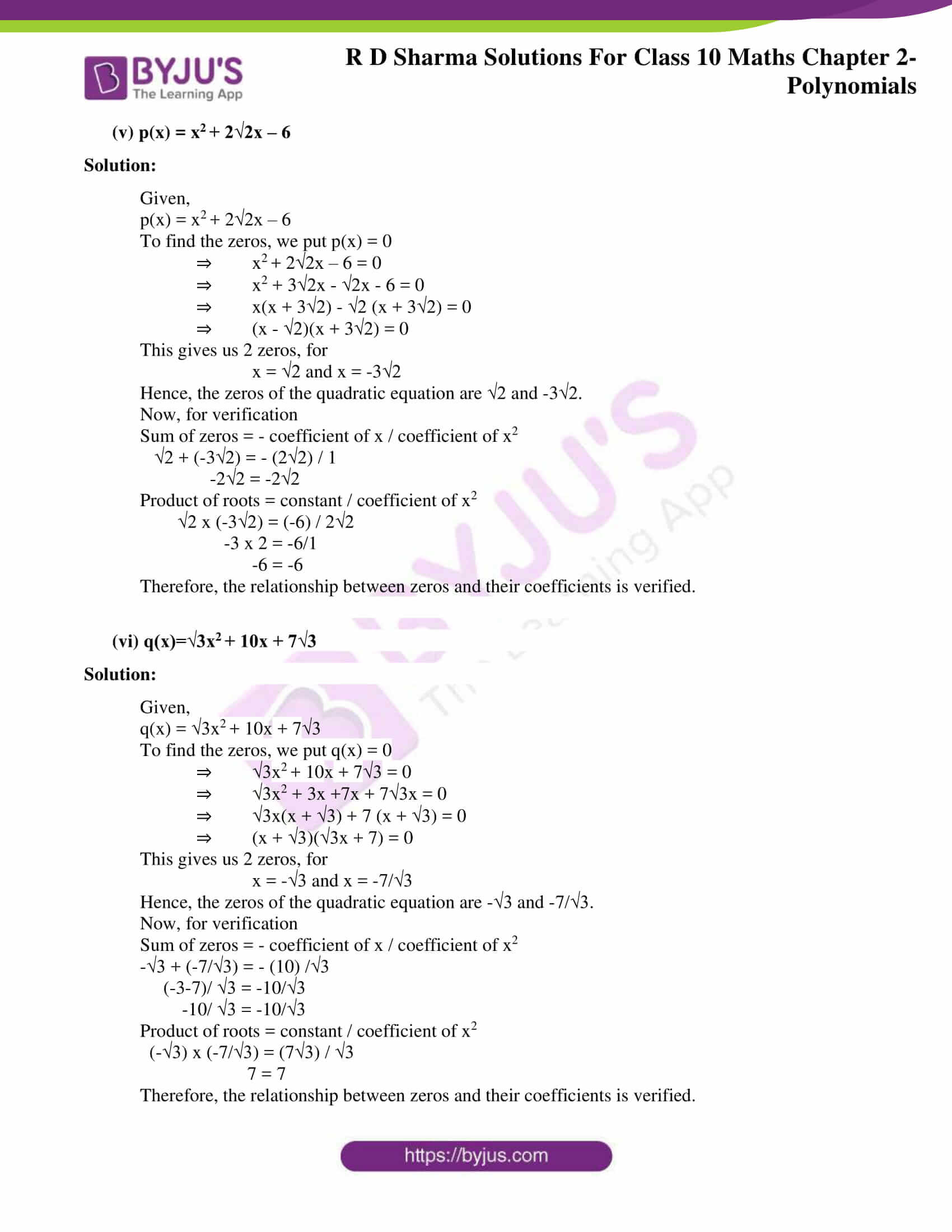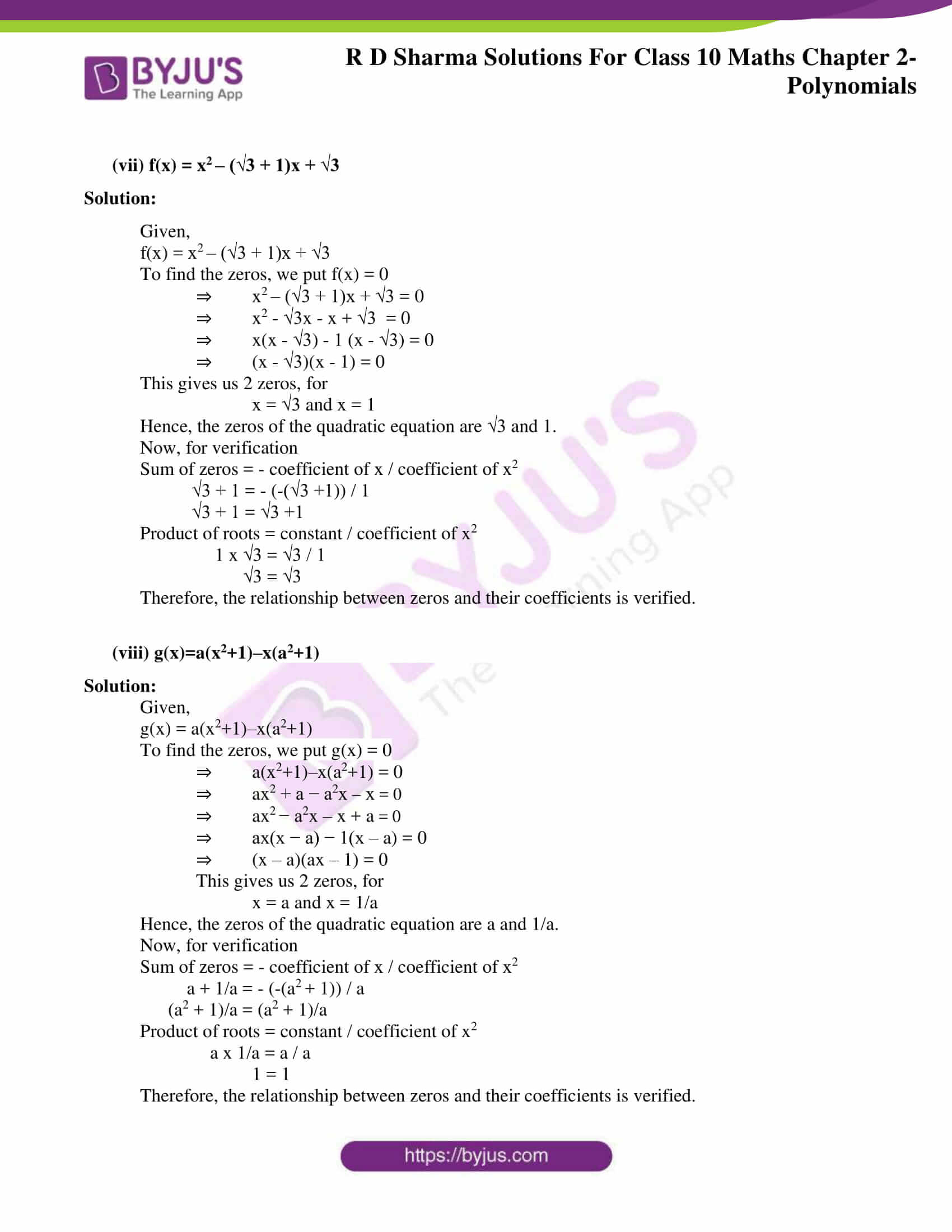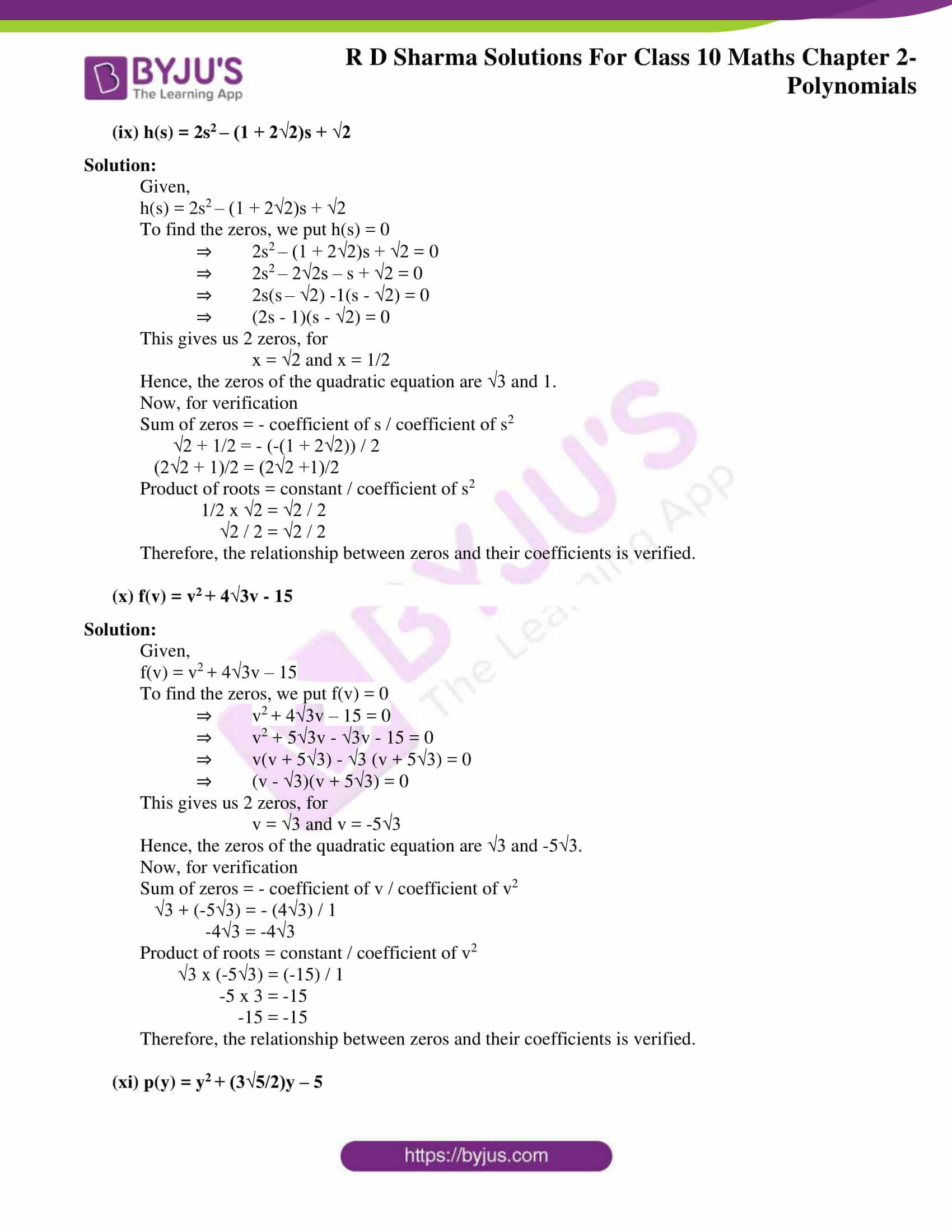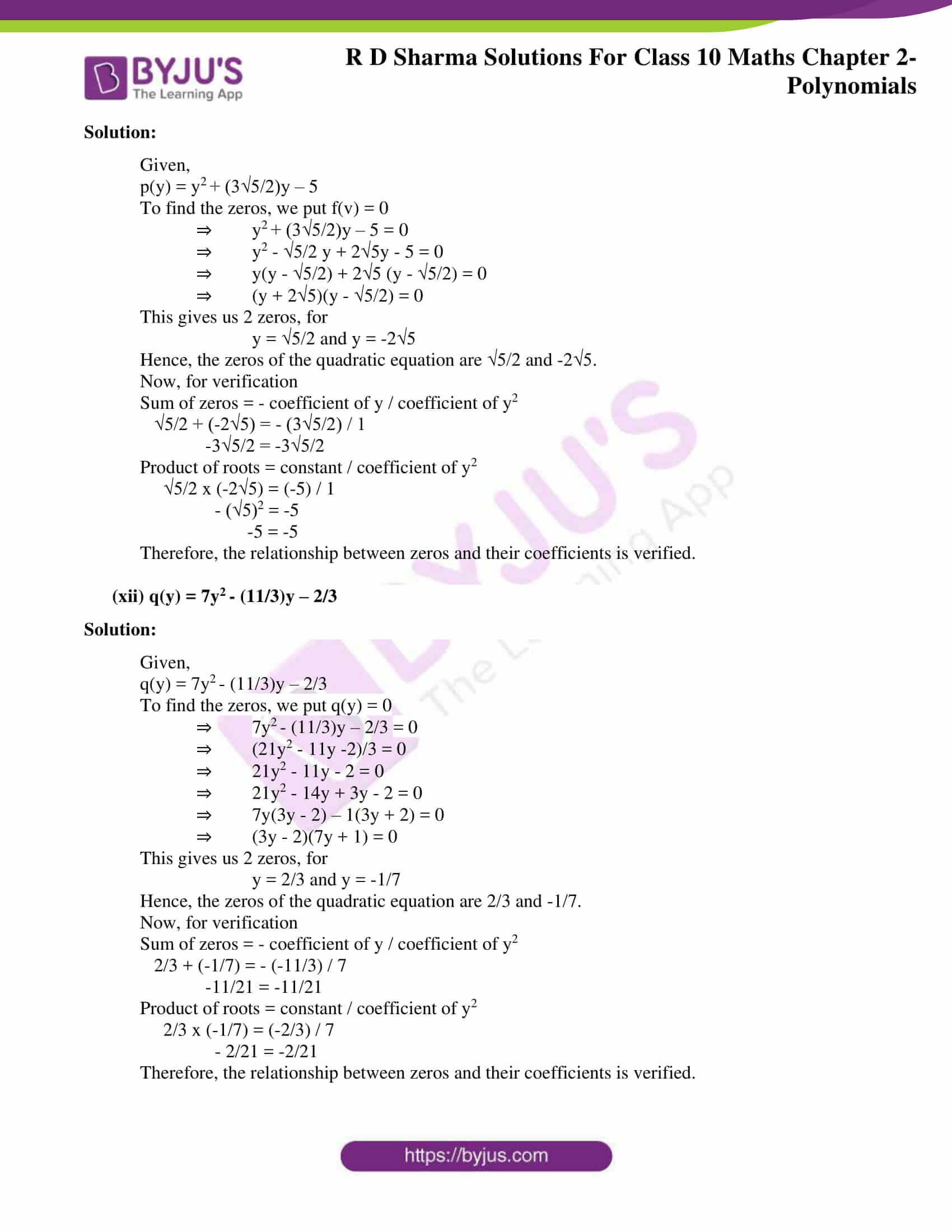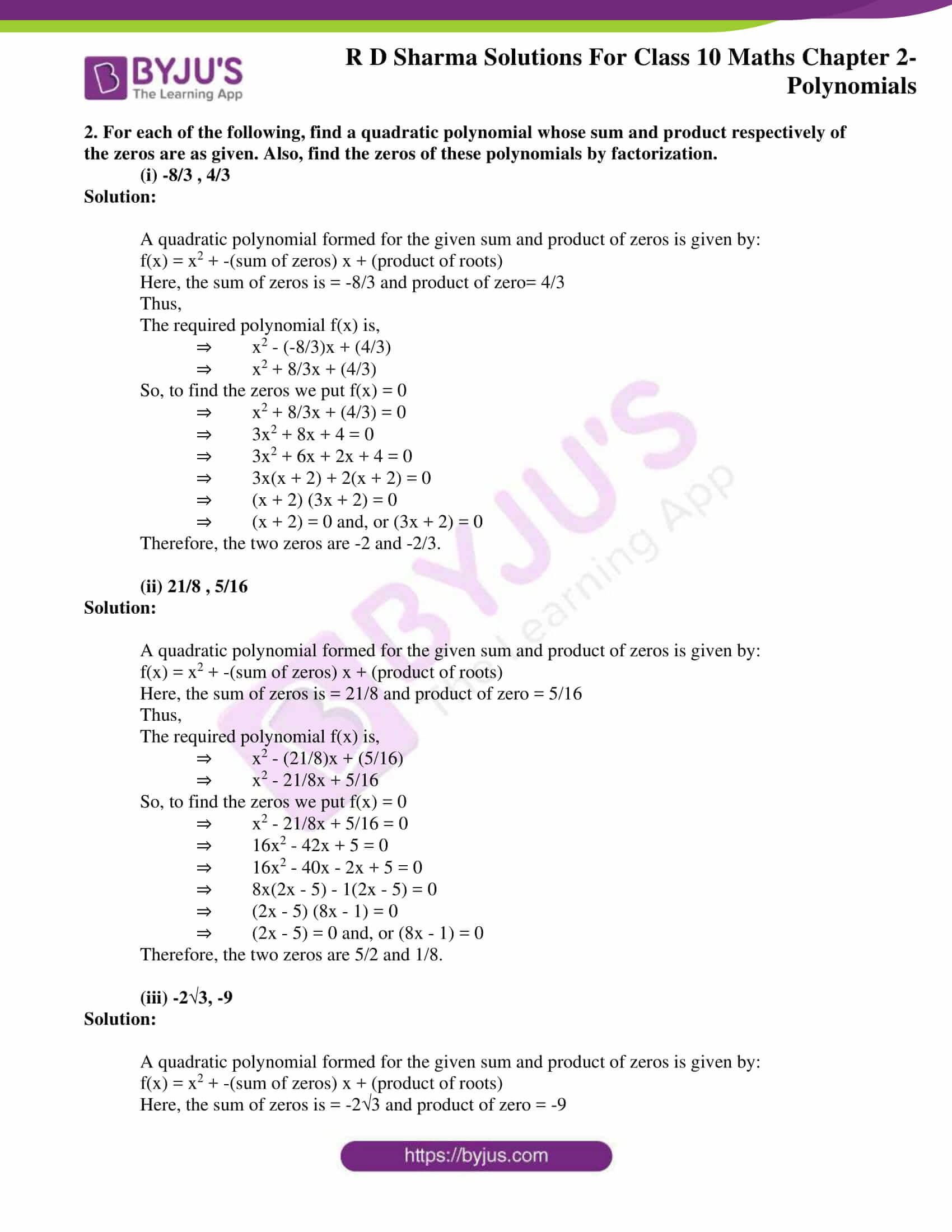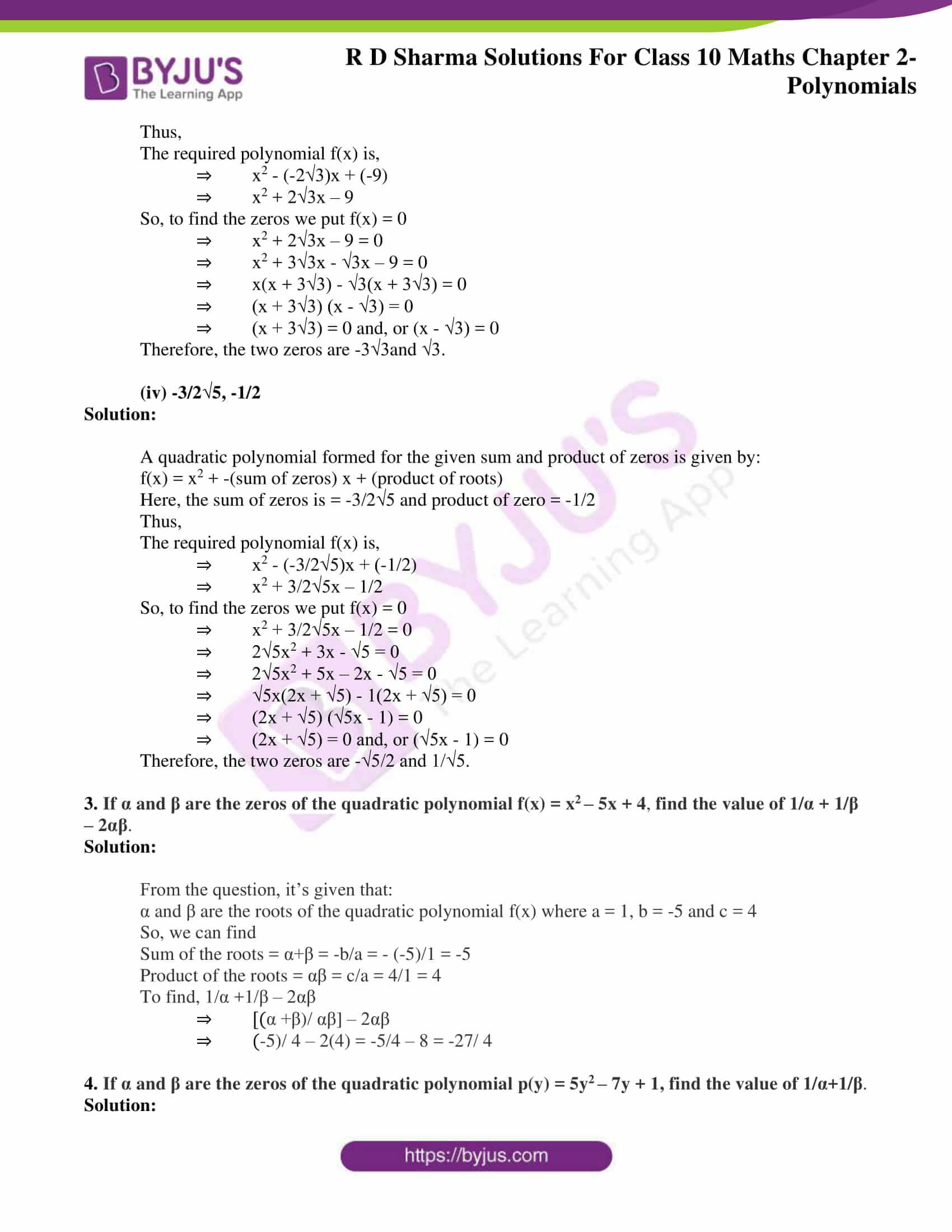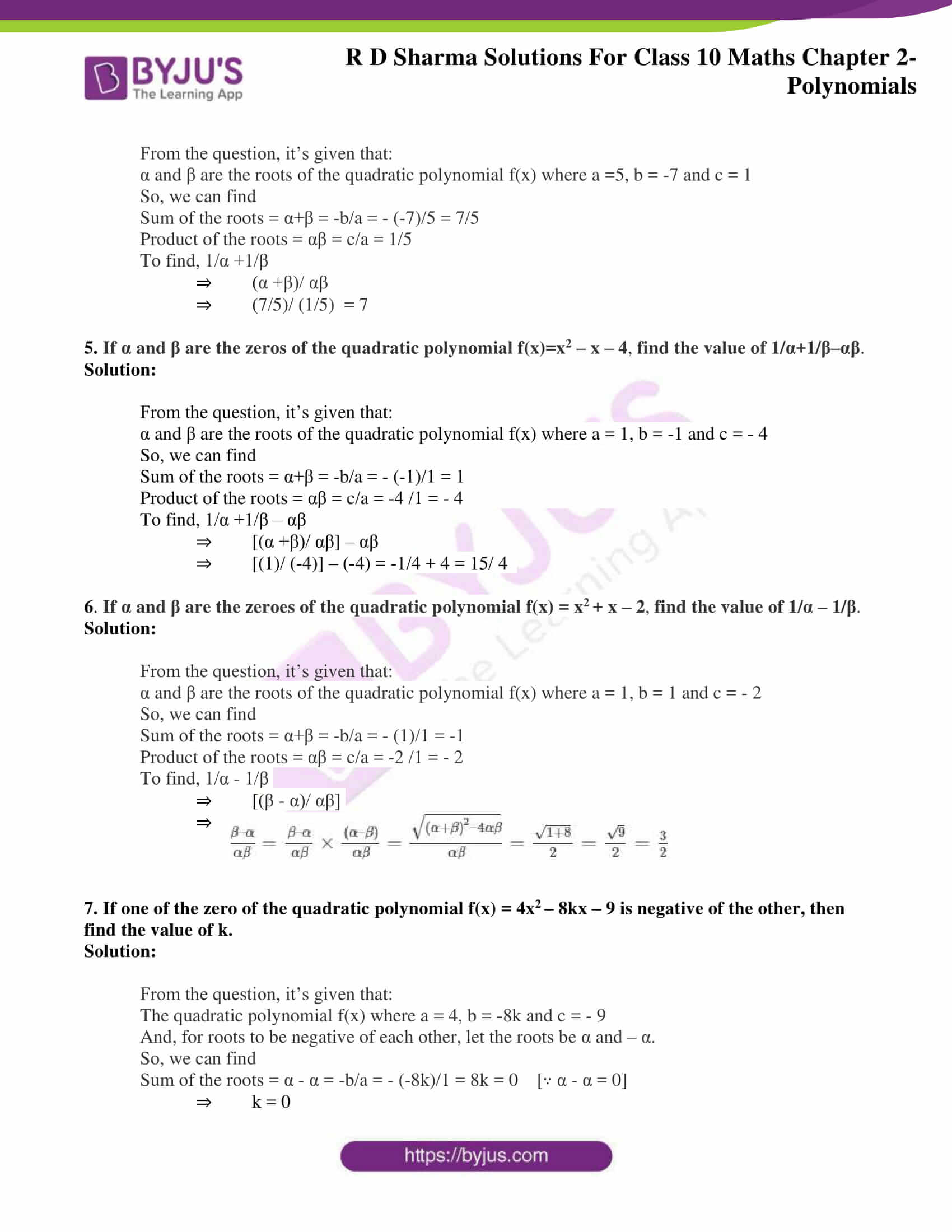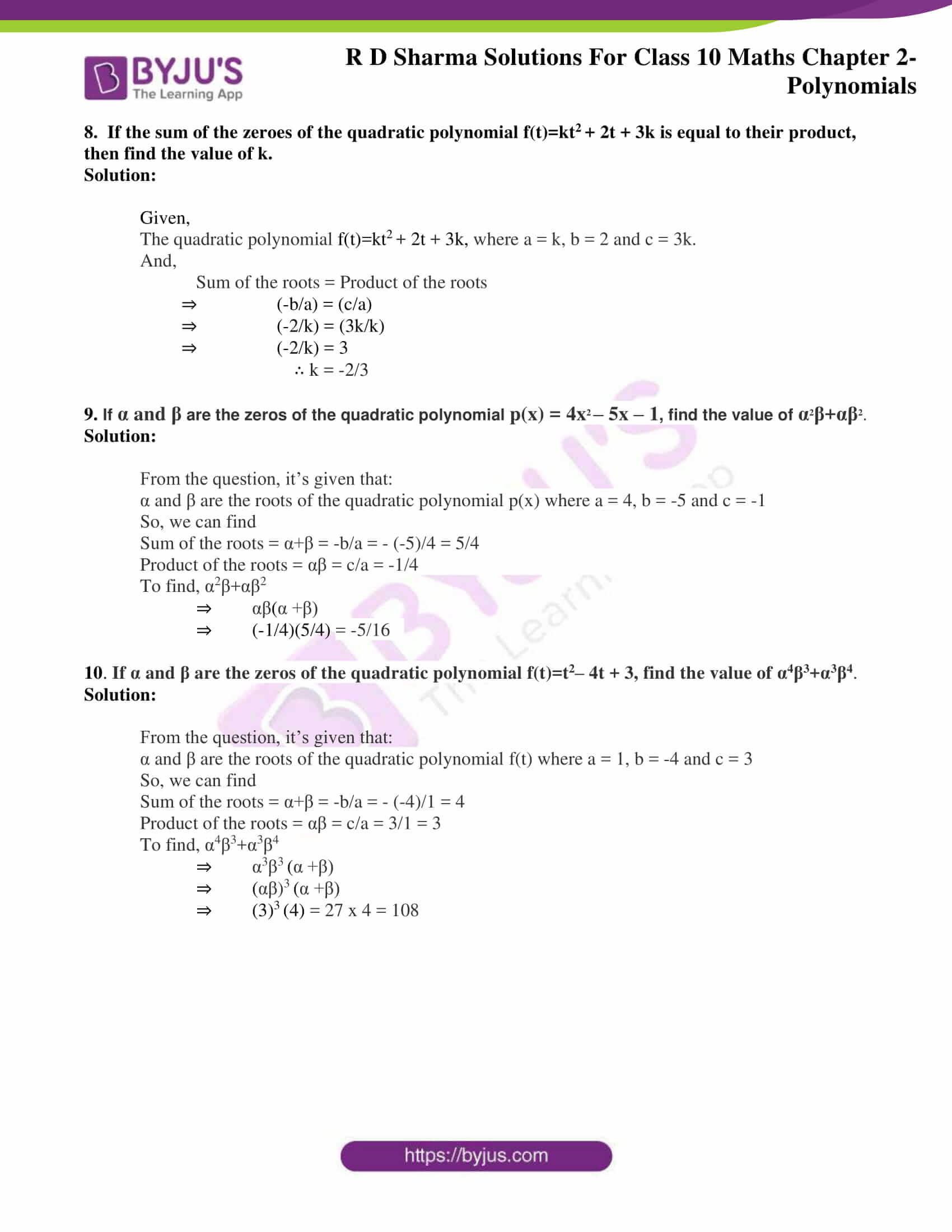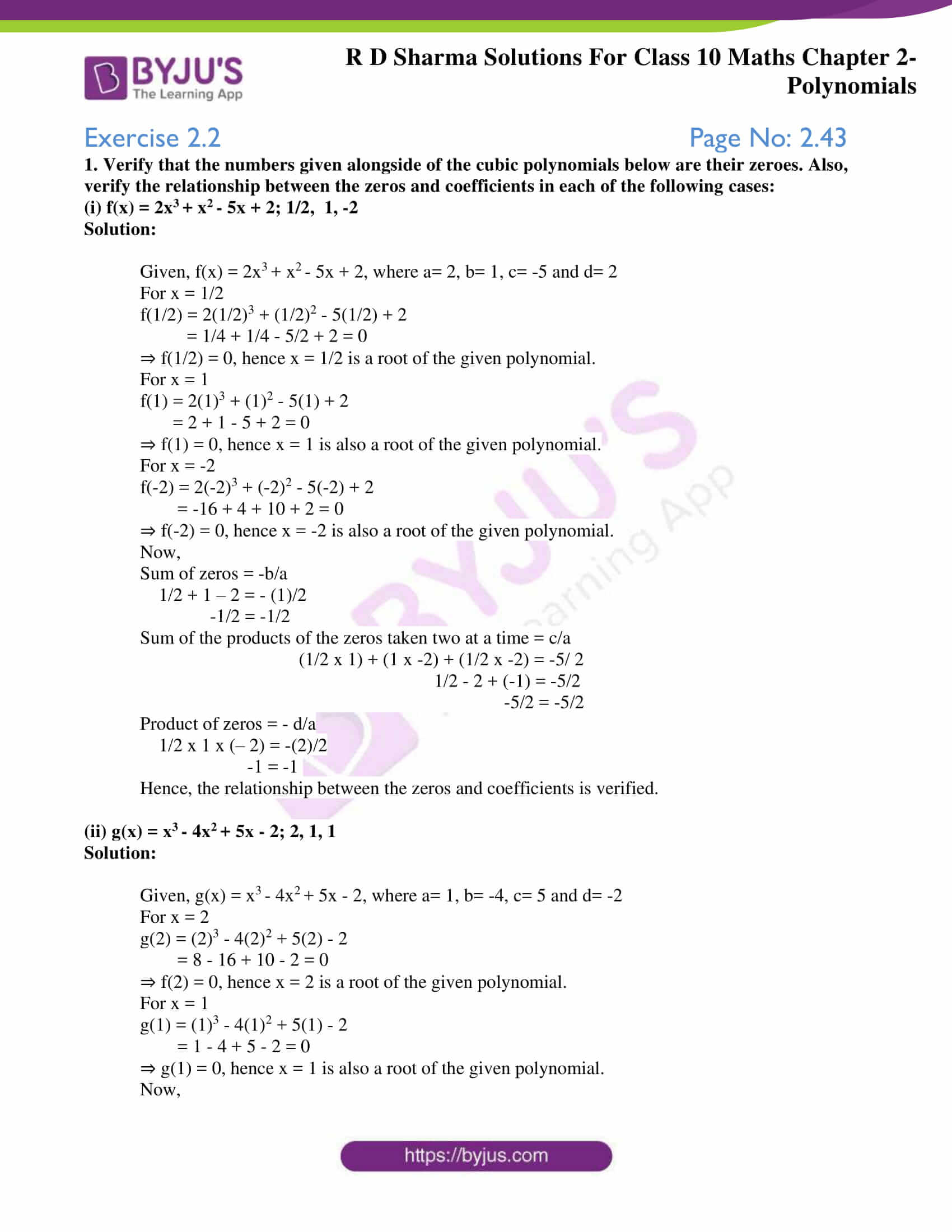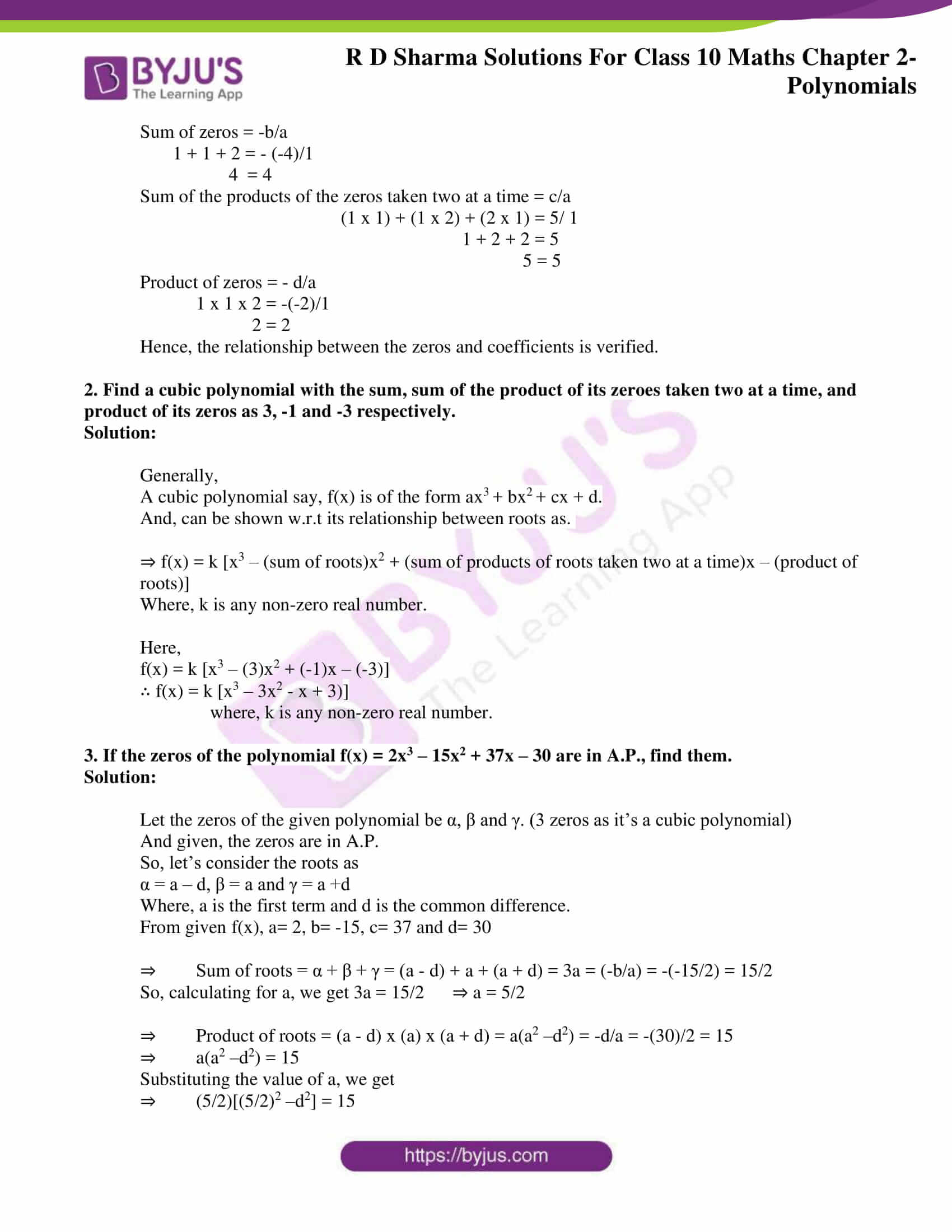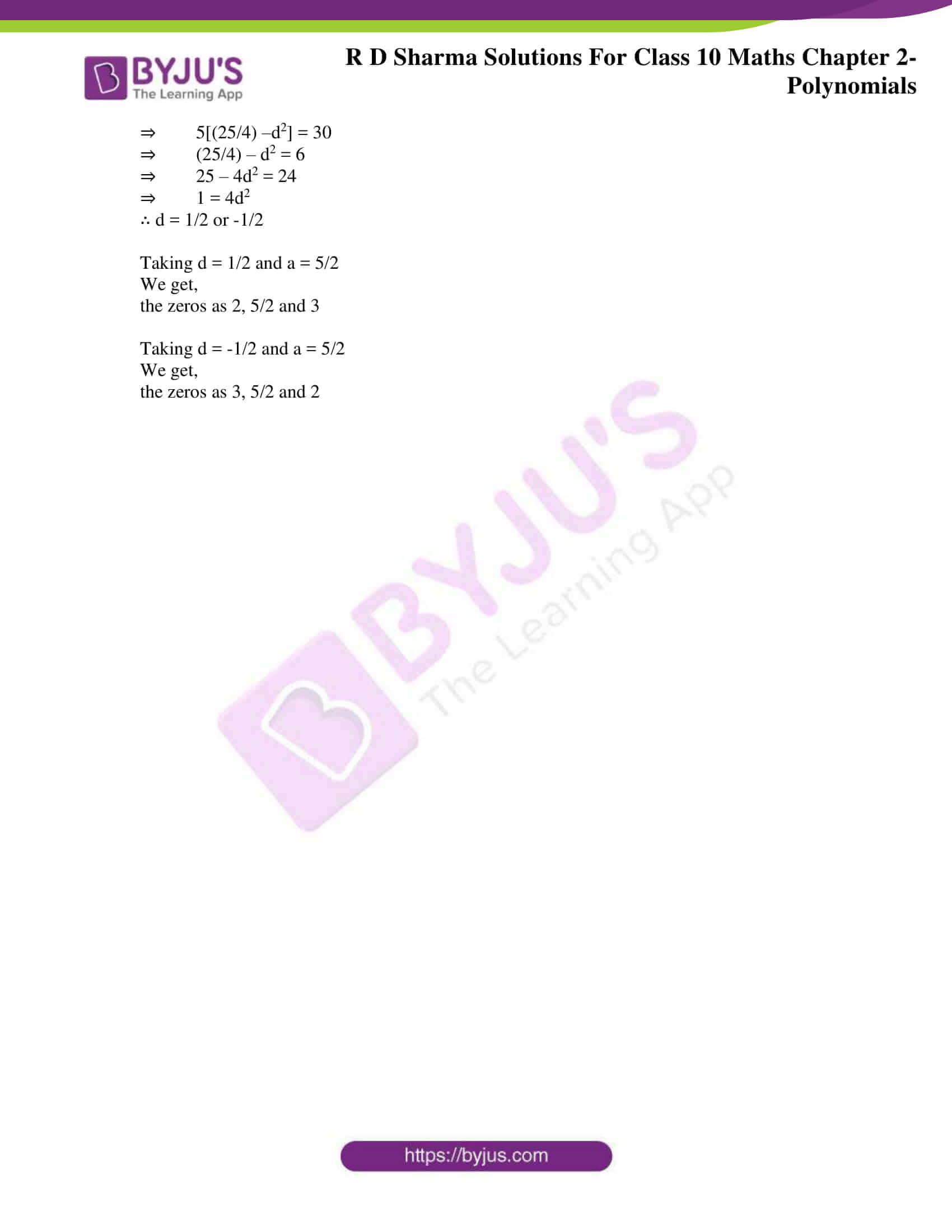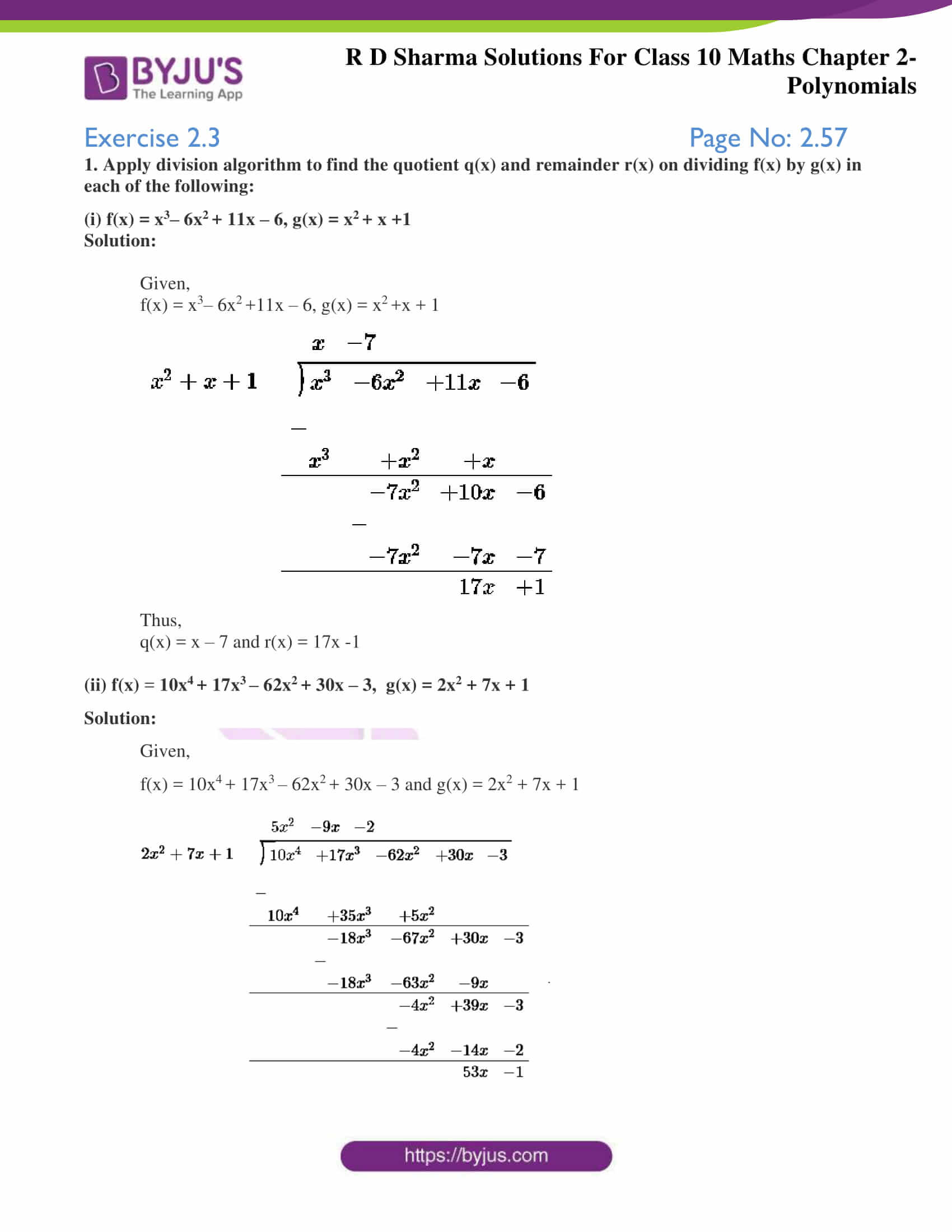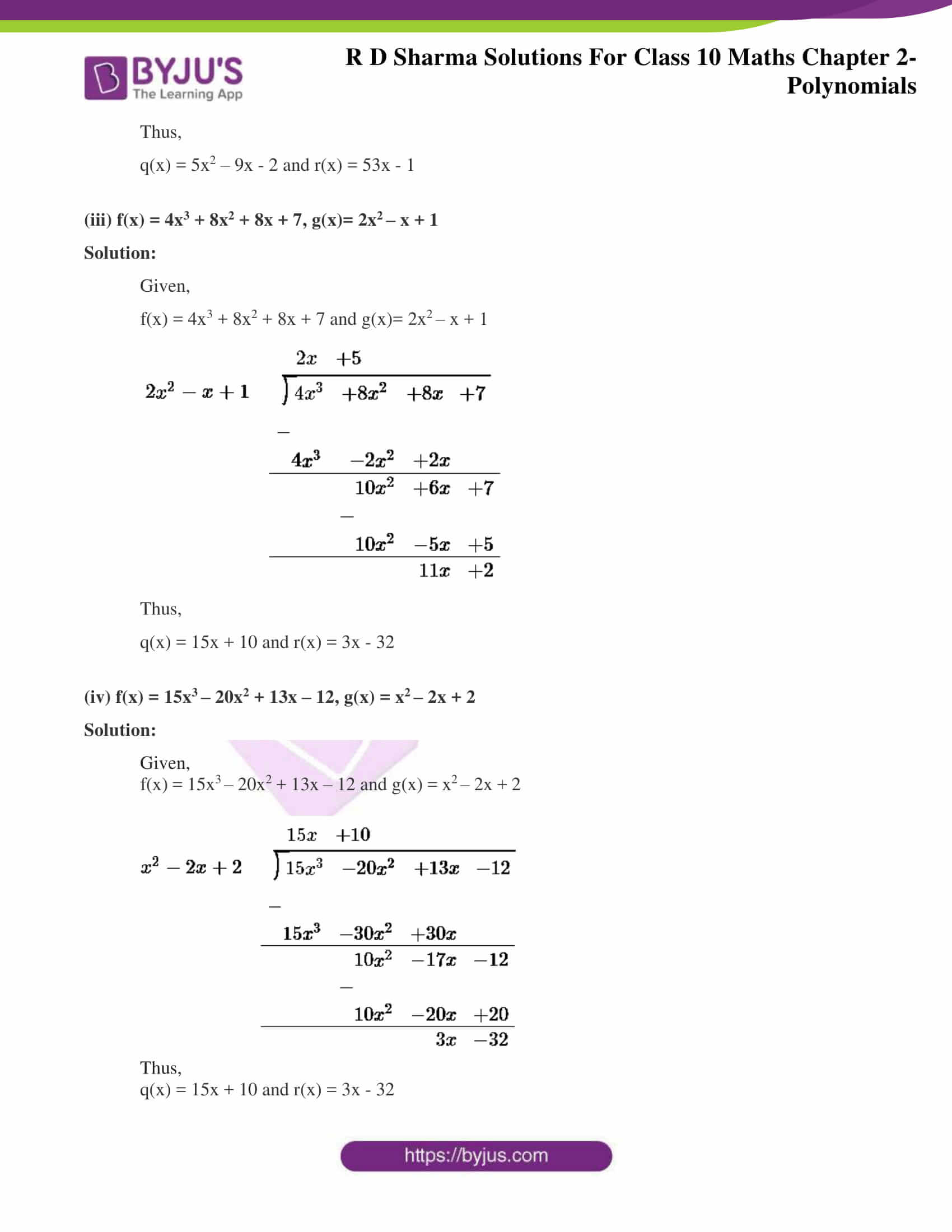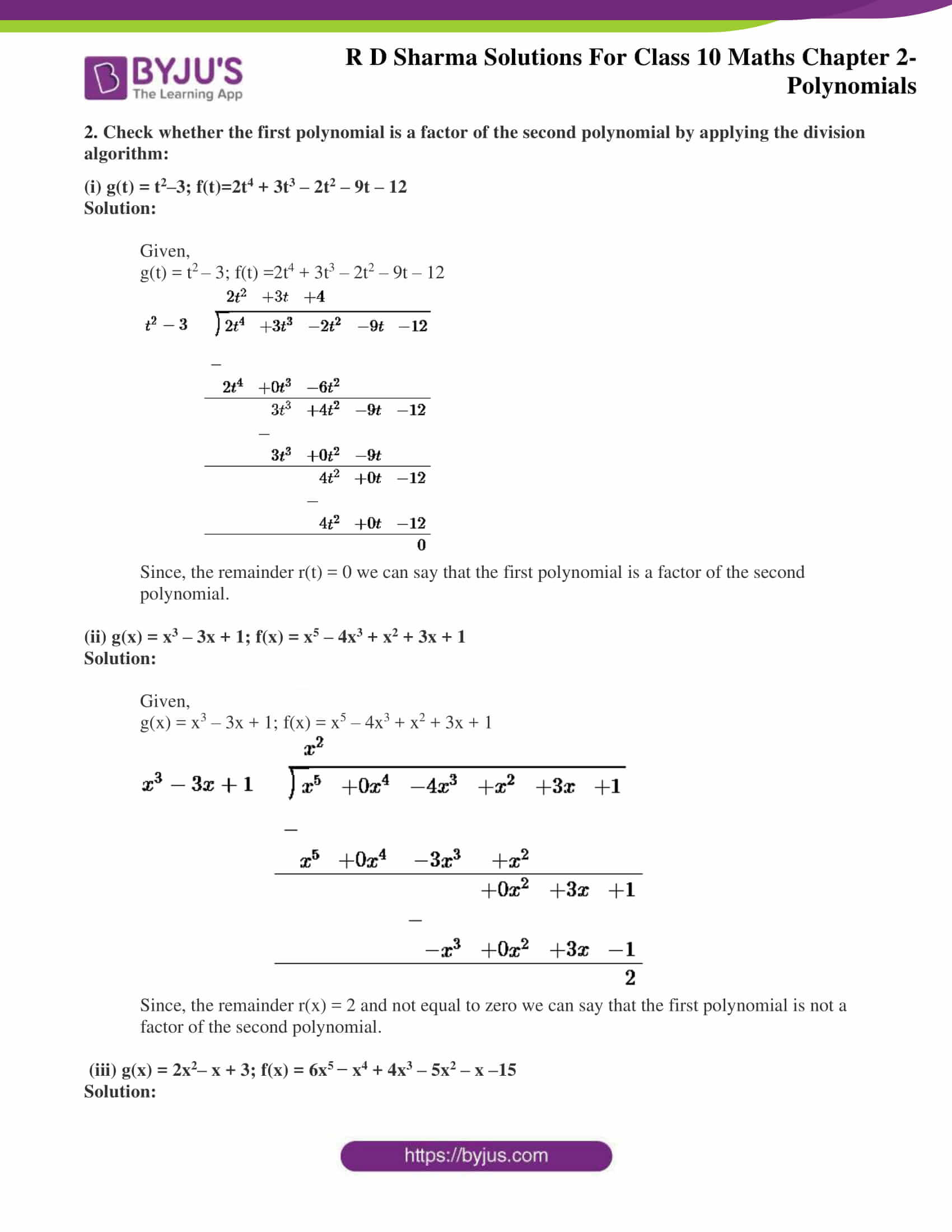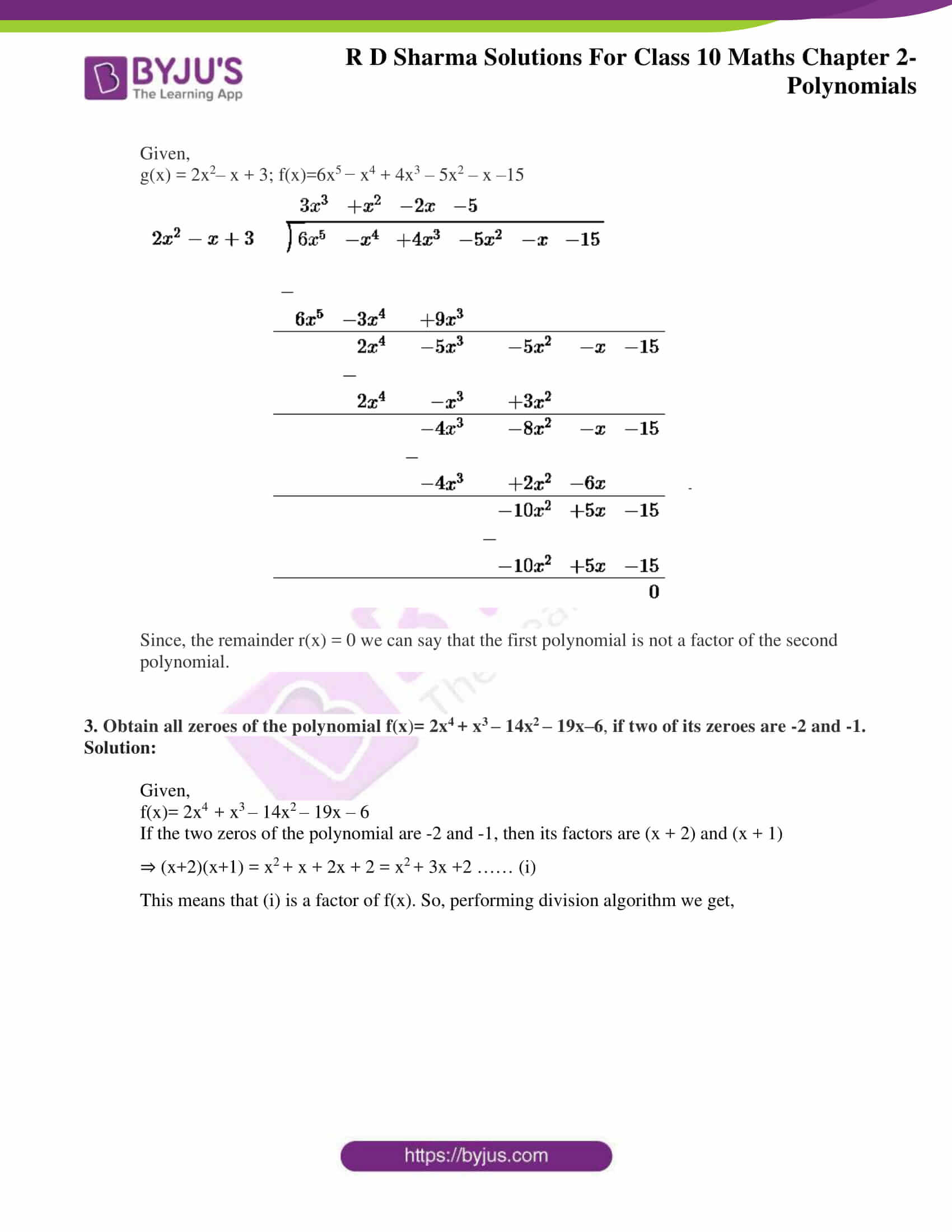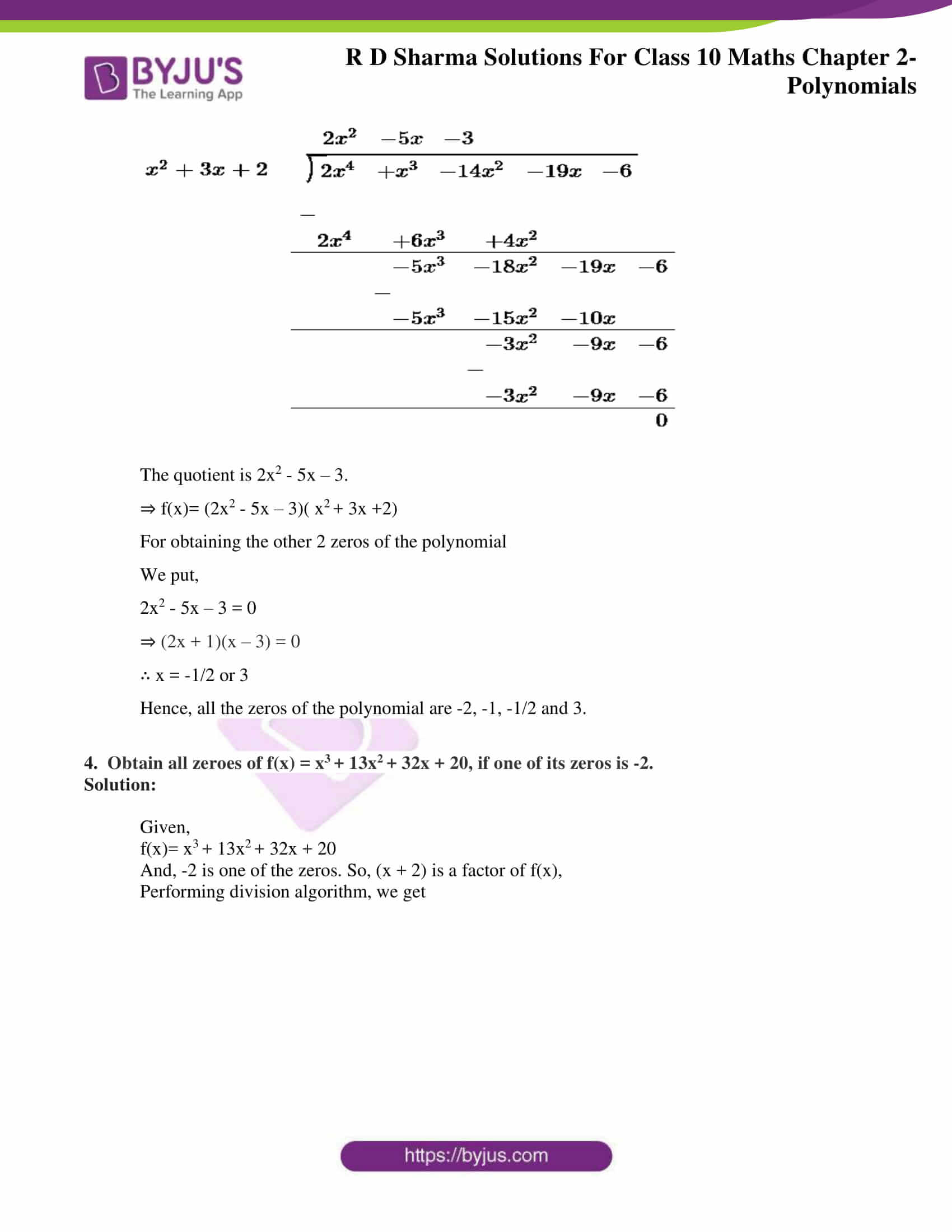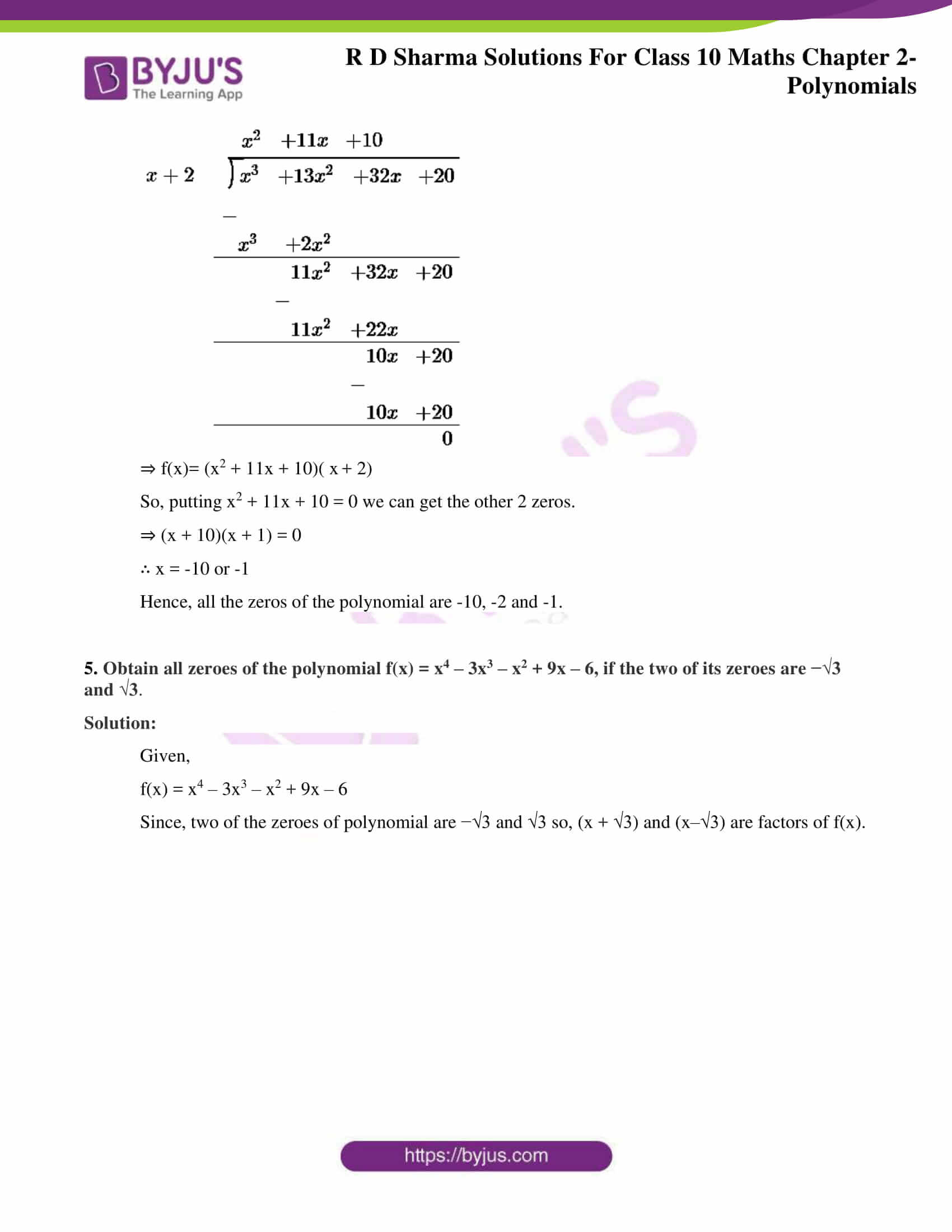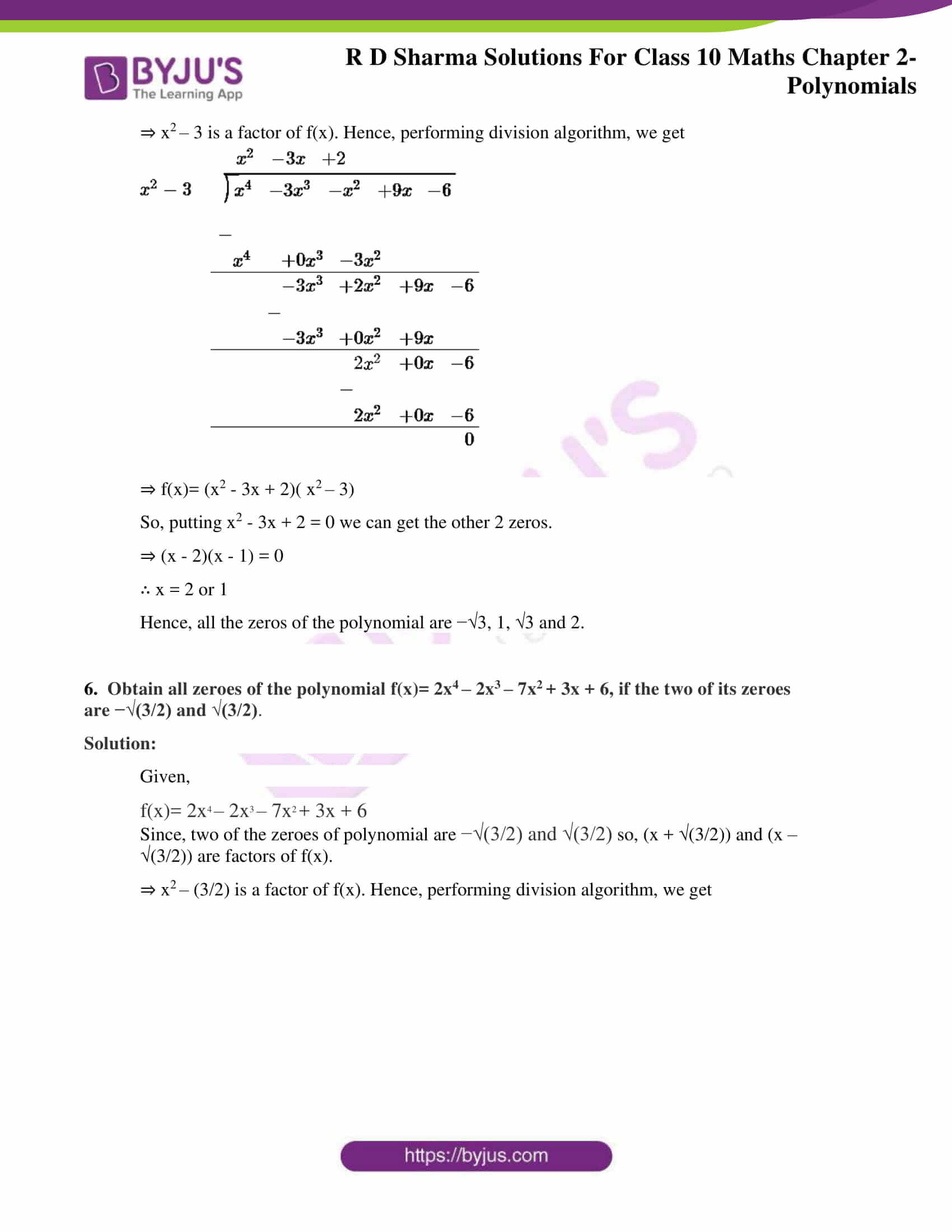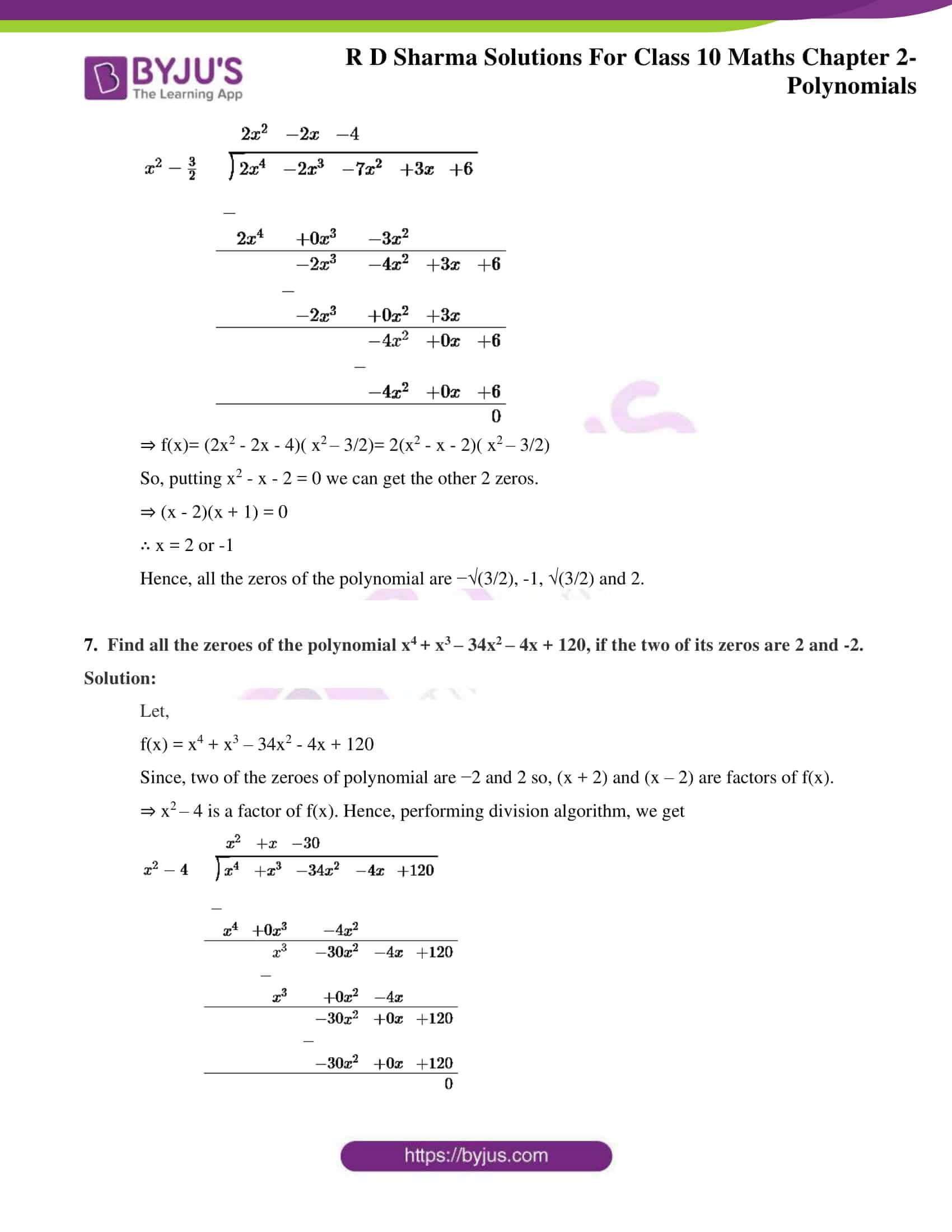Chapter 2- Polynomials has three exercises and RD Sharma Solutions for Class 10 here contains the answers to the problems done in a very intelligible and detailed manner. Let’s get an insight to this chapter to get a better idea of what it’s about.

• Polynomial and its types
• Geometrical representation of linear and quadratic polynomials
• The geometric meaning of the zeros of a polynomial
• Relationship between the zeros and coefficients of a polynomial

### RD Sharma class 10 Chapter 2 Exercise 2.1 Page No: 2.33

1. Find the zeros of each of the following quadratic polynomials and verify the relationship between the zeros and their coefficients:

(i) f(x) = x– 2x – 8

Solution:

Given,

f(x) = x– 2x – 8

To find the zeros, we put f(x) = 0

⇒ x– 2x – 8 = 0

⇒  x2 – 4x + 2x – 8 = 0

⇒ x(x – 4) + 2(x – 4) = 0

⇒ (x – 4)(x + 2) = 0

This gives us 2 zeros, for

x = 4 and x = -2

Hence, the zeros of the quadratic equation are 4 and -2.

Now, for verification

Sum of zeros = – coefficient of x / coefficient of x2

4 + (-2)= – (-2) / 1

2 = 2

Product of roots = constant / coefficient of x2

4 x (-2) = (-8) / 1

-8 = -8

Therefore, the relationship between zeros and their coefficients is verified.

(ii) g(s) = 4s– 4s + 1

Solution:

Given,

g(s) = 4s– 4s + 1

To find the zeros, we put g(s) = 0

⇒ 4s– 4s + 1 = 0

⇒  4s2 – 2s – 2s + 1= 0

⇒  2s(2s – 1) – (2s – 1) = 0

⇒ (2s – 1)(2s – 1) = 0

This gives us 2 zeros, for

s = 1/2 and s = 1/2

Hence, the zeros of the quadratic equation are 1/2 and 1/2.

Now, for verification

Sum of zeros = – coefficient of s / coefficient of s2

1/2 + 1/2 = – (-4) / 4

1 = 1

Product of roots = constant / coefficient of s2

1/2 x 1/2 = 1/4

1/4 = 1/4

Therefore, the relationship between zeros and their coefficients is verified.

(iii) h(t)=t– 15

Solution:

Given,

h(t) = t– 15 = t+(0)t – 15

To find the zeros, we put h(t) = 0

⇒ t– 15 = 0

⇒ (t + √15)(t – √15)= 0

This gives us 2 zeros, for

t = √15 and t = -√15

Hence, the zeros of the quadratic equation are √15 and -√15.

Now, for verification

Sum of zeros = – coefficient of t / coefficient of t2

√15 + (-√15) = – (0) / 1

0 = 0

Product of roots = constant / coefficient of t2

√15 x (-√15) = -15/1

-15 = -15

Therefore, the relationship between zeros and their coefficients is verified.

(iv) f(x) = 6x2 – 3 – 7x

Solution:

Given,

f(x) = 6x– 3 – 7x

To find the zeros, we put f(x) = 0

⇒ 6x– 3 – 7x = 0

⇒  6x2 – 9x + 2x – 3 = 0

⇒ 3x(2x – 3) + 1(2x – 3) = 0

⇒ (2x – 3)(3x + 1) = 0

This gives us 2 zeros, for

x = 3/2 and x = -1/3

Hence, the zeros of the quadratic equation are 3/2 and -1/3.

Now, for verification

Sum of zeros = – coefficient of x / coefficient of x2

3/2 + (-1/3) = – (-7) / 6

7/6 = 7/6

Product of roots = constant / coefficient of x2

3/2 x (-1/3) = (-3) / 6

-1/2 = -1/2

Therefore, the relationship between zeros and their coefficients is verified.

(v) p(x) = x+ 2√2x – 6

Solution:

Given,

p(x) = x+ 2√2x – 6

To find the zeros, we put p(x) = 0

⇒ x+ 2√2x – 6 = 0

⇒  x2 + 3√2x – √2x – 6 = 0

⇒ x(x + 3√2) – √2 (x + 3√2) = 0

⇒ (x – √2)(x + 3√2) = 0

This gives us 2 zeros, for

x = √2 and x = -3√2

Hence, the zeros of the quadratic equation are √2 and -3√2.

Now, for verification

Sum of zeros = – coefficient of x / coefficient of x2

√2 + (-3√2) = – (2√2) / 1

-2√2 = -2√2

Product of roots = constant / coefficient of x2

√2 x (-3√2) = (-6) / 2√2

-3 x 2 = -6/1

-6 = -6

Therefore, the relationship between zeros and their coefficients is verified.

(vi) q(x)=√3x+ 10x + 7√3

Solution:

Given,

q(x) = √3x+ 10x + 7√3

To find the zeros, we put q(x) = 0

⇒ √3x+ 10x + 7√3 = 0

⇒  √3x2 + 3x +7x + 7√3x = 0

⇒ √3x(x + √3) + 7 (x + √3) = 0

⇒ (x + √3)(√3x + 7) = 0

This gives us 2 zeros, for

x = -√3 and x = -7/√3

Hence, the zeros of the quadratic equation are -√3 and -7/√3.

Now, for verification

Sum of zeros = – coefficient of x / coefficient of x2

-√3 + (-7/√3) = – (10) /√3

(-3-7)/ √3 = -10/√3

-10/ √3 = -10/√3

Product of roots = constant / coefficient of x2

(-√3) x (-7/√3) = (7√3) / √3

7 = 7

Therefore, the relationship between zeros and their coefficients is verified.

(vii) f(x) = x– (√3 + 1)x + √3

Solution:

Given,

f(x) = x– (√3 + 1)x + √3

To find the zeros, we put f(x) = 0

⇒ x– (√3 + 1)x + √3 = 0

⇒  x2 – √3x – x + √3 = 0

⇒ x(x – √3) – 1 (x – √3) = 0

⇒ (x – √3)(x – 1) = 0

This gives us 2 zeros, for

x = √3 and x = 1

Hence, the zeros of the quadratic equation are √3 and 1.

Now, for verification

Sum of zeros = – coefficient of x / coefficient of x2

√3 + 1 = – (-(√3 +1)) / 1

√3 + 1 = √3 +1

Product of roots = constant / coefficient of x2

1 x √3 = √3 / 1

√3 = √3

Therefore, the relationship between zeros and their coefficients is verified.

(viii) g(x)=a(x2+1)–x(a2+1)

Solution:

Given,

g(x) = a(x2+1)–x(a2+1)

To find the zeros, we put g(x) = 0

⇒ a(x2+1)–x(a2+1) = 0

⇒ ax2 + a − a2x – x = 0

⇒ ax− a2x – x + a = 0

⇒ ax(x − a) − 1(x – a) = 0

⇒ (x – a)(ax – 1) = 0

This gives us 2 zeros, for

x = a and x = 1/a

Hence, the zeros of the quadratic equation are a and 1/a.

Now, for verification

Sum of zeros = – coefficient of x / coefficient of x2

a + 1/a = – (-(a2 + 1)) / a

(a2 + 1)/a = (a2 + 1)/a

Product of roots = constant / coefficient of x2

a x 1/a = a / a

1 = 1

Therefore, the relationship between zeros and their coefficients is verified.

(ix) h(s) = 2s– (1 + 2√2)s + √2

Solution:

Given,

h(s) = 2s– (1 + 2√2)s + √2

To find the zeros, we put h(s) = 0

⇒ 2s– (1 + 2√2)s + √2 = 0

⇒  2s– 2√2s – s + √2 = 0

⇒ 2s(s – √2) -1(s – √2) = 0

⇒ (2s – 1)(s – √2) = 0

This gives us 2 zeros, for

x = √2 and x = 1/2

Hence, the zeros of the quadratic equation are √3 and 1.

Now, for verification

Sum of zeros = – coefficient of s / coefficient of s2

√2 + 1/2 = – (-(1 + 2√2)) / 2

(2√2 + 1)/2 = (2√2 +1)/2

Product of roots = constant / coefficient of s2

1/2 x √2 = √2 / 2

√2 / 2 = √2 / 2

Therefore, the relationship between zeros and their coefficients is verified.

(x) f(v) = v+ 4√3v – 15

Solution:

Given,

f(v) = v+ 4√3v – 15

To find the zeros, we put f(v) = 0

⇒ v+ 4√3v – 15 = 0

⇒  v2 + 5√3v – √3v – 15 = 0

⇒ v(v + 5√3) – √3 (v + 5√3) = 0

⇒ (v – √3)(v + 5√3) = 0

This gives us 2 zeros, for

v = √3 and v = -5√3

Hence, the zeros of the quadratic equation are √3 and -5√3.

Now, for verification

Sum of zeros = – coefficient of v / coefficient of v2

√3 + (-5√3) = – (4√3) / 1

-4√3 = -4√3

Product of roots = constant / coefficient of v2

√3 x (-5√3) = (-15) / 1

-5 x 3 = -15

-15 = -15

Therefore, the relationship between zeros and their coefficients is verified.

(xi) p(y) = y+ (3√5/2)y – 5

Solution:

Given,

p(y) = y+ (3√5/2)y – 5

To find the zeros, we put f(v) = 0

⇒ y+ (3√5/2)y – 5 = 0

⇒  y2 – √5/2 y + 2√5y – 5 = 0

⇒ y(y – √5/2) + 2√5 (y – √5/2) = 0

⇒ (y + 2√5)(y – √5/2) = 0

This gives us 2 zeros, for

y = √5/2 and y = -2√5

Hence, the zeros of the quadratic equation are √5/2 and -2√5.

Now, for verification

Sum of zeros = – coefficient of y / coefficient of y2

√5/2 + (-2√5) = – (3√5/2) / 1

-3√5/2 = -3√5/2

Product of roots = constant / coefficient of y2

√5/2 x (-2√5) = (-5) / 1

– (√5)2 = -5

-5 = -5

Therefore, the relationship between zeros and their coefficients is verified.

(xii) q(y) = 7y– (11/3)y – 2/3

Solution:

Given,

q(y) = 7y– (11/3)y – 2/3

To find the zeros, we put q(y) = 0

⇒ 7y– (11/3)y – 2/3 = 0

⇒  (21y2 – 11y -2)/3 = 0

⇒ 21y2 – 11y – 2 = 0

⇒ 21y2 – 14y + 3y – 2 = 0

⇒ 7y(3y – 2) – 1(3y + 2) = 0

⇒ (3y – 2)(7y + 1) = 0

This gives us 2 zeros, for

y = 2/3 and y = -1/7

Hence, the zeros of the quadratic equation are 2/3 and -1/7.

Now, for verification

Sum of zeros = – coefficient of y / coefficient of y2

2/3 + (-1/7) = – (-11/3) / 7

-11/21 = -11/21

Product of roots = constant / coefficient of y2

2/3 x (-1/7) = (-2/3) / 7

– 2/21 = -2/21

Therefore, the relationship between zeros and their coefficients is verified.

2. For each of the following, find a quadratic polynomial whose sum and product respectively of the zeros are as given. Also, find the zeros of these polynomials by factorization.

(i) -8/3 , 4/3

Solution:

A quadratic polynomial formed for the given sum and product of zeros is given by:

f(x) = x2 + -(sum of zeros) x + (product of roots)

Here, the sum of zeros is = -8/3 and product of zero= 4/3

Thus,

The required polynomial f(x) is,

⇒ x2 – (-8/3)x + (4/3)

⇒ x2 + 8/3x + (4/3)

So, to find the zeros we put f(x) = 0

⇒ x2 + 8/3x + (4/3) = 0

⇒ 3x2 + 8x + 4 = 0

⇒ 3x2 + 6x + 2x + 4 = 0

⇒ 3x(x + 2) + 2(x + 2) = 0

⇒ (x + 2) (3x + 2) = 0

⇒ (x + 2) = 0 and, or (3x + 2) = 0

Therefore, the two zeros are -2 and -2/3.

(ii) 21/8 , 5/16

Solution:

A quadratic polynomial formed for the given sum and product of zeros is given by:

f(x) = x2 + -(sum of zeros) x + (product of roots)

Here, the sum of zeros is = 21/8 and product of zero = 5/16

Thus,

The required polynomial f(x) is,

⇒ x2 – (21/8)x + (5/16)

⇒ x2 – 21/8x + 5/16

So, to find the zeros we put f(x) = 0

⇒ x2 – 21/8x + 5/16 = 0

⇒ 16x2 – 42x + 5 = 0

⇒ 16x2 – 40x – 2x + 5 = 0

⇒ 8x(2x – 5) – 1(2x – 5) = 0

⇒ (2x – 5) (8x – 1) = 0

⇒ (2x – 5) = 0 and, or (8x – 1) = 0

Therefore, the two zeros are 5/2 and 1/8.

(iii) -2√3, -9

Solution:

A quadratic polynomial formed for the given sum and product of zeros is given by:

f(x) = x2 + -(sum of zeros) x + (product of roots)

Here, the sum of zeros is = -2√3 and product of zero = -9

Thus,

The required polynomial f(x) is,

⇒ x2 – (-2√3)x + (-9)

⇒ x2 + 2√3x – 9

So, to find the zeros we put f(x) = 0

⇒ x2 + 2√3x – 9 = 0

⇒ x2 + 3√3x – √3x – 9 = 0

⇒ x(x + 3√3) – √3(x + 3√3) = 0

⇒ (x + 3√3) (x – √3) = 0

⇒ (x + 3√3) = 0 and, or (x – √3) = 0

Therefore, the two zeros are -3√3and √3.

(iv) -3/2√5, -1/2

Solution:

A quadratic polynomial formed for the given sum and product of zeros is given by:

f(x) = x2 + -(sum of zeros) x + (product of roots)

Here, the sum of zeros is = -3/2√5 and product of zero = -1/2

Thus,

The required polynomial f(x) is,

⇒ x2 – (-3/2√5)x + (-1/2)

⇒ x2 + 3/2√5x – 1/2

So, to find the zeros we put f(x) = 0

⇒ x2 + 3/2√5x – 1/2 = 0

⇒ 2√5x2 + 3x – √5 = 0

⇒ 2√5x2 + 5x – 2x – √5 = 0

⇒ √5x(2x + √5) – 1(2x + √5) = 0

⇒ (2x + √5) (√5x – 1) = 0

⇒ (2x + √5) = 0 and, or (√5x – 1) = 0

Therefore, the two zeros are -√5/2 and 1/√5.

3. If α and β are the zeros of the quadratic polynomial f(x) = x2 – 5x + 4, find the value of 1/α + 1/β – 2αβ.

Solution:

From the question, it’s given that:

α and β are the roots of the quadratic polynomial f(x) where a = 1, b = -5 and c = 4

So, we can find

Sum of the roots = α+β = -b/a = – (-5)/1 = -5

Product of the roots = αβ = c/a = 4/1 = 4

To find, 1/α +1/β – 2αβ

⇒ [(α +β)/ αβ] – 2αβ

⇒ (-5)/ 4 – 2(4) = -5/4 – 8 = -27/ 4

4. If α and β are the zeros of the quadratic polynomial p(y) = 5y2 – 7y + 1, find the value of 1/α+1/β.

Solution:

From the question, it’s given that:

α and β are the roots of the quadratic polynomial f(x) where a =5, b = -7 and c = 1

So, we can find

Sum of the roots = α+β = -b/a = – (-7)/5 = 7/5

Product of the roots = αβ = c/a = 1/5

To find, 1/α +1/β

⇒ (α +β)/ αβ

⇒ (7/5)/ (1/5) = 7

5. If α and β are the zeros of the quadratic polynomial f(x)=x2 – x – 4, find the value of 1/α+1/β–αβ.

Solution:

From the question, it’s given that:

α and β are the roots of the quadratic polynomial f(x) where a = 1, b = -1 and c = – 4

So, we can find

Sum of the roots = α+β = -b/a = – (-1)/1 = 1

Product of the roots = αβ = c/a = -4 /1 = – 4

To find, 1/α +1/β – αβ

⇒ [(α +β)/ αβ] – αβ

⇒ [(1)/ (-4)] – (-4) = -1/4 + 4 = 15/ 4

6. If α and β are the zeroes of the quadratic polynomial f(x) = x2 + x – 2, find the value of 1/α – 1/β.

Solution:

From the question, it’s given that:

α and β are the roots of the quadratic polynomial f(x) where a = 1, b = 1 and c = – 2

So, we can find

Sum of the roots = α+β = -b/a = – (1)/1 = -1

Product of the roots = αβ = c/a = -2 /1 = – 2

To find, 1/α – 1/β

⇒ [(β – α)/ αβ]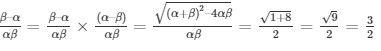7. If one of the zero of the quadratic polynomial f(x) = 4x2 – 8kx – 9 is negative of the other, then find the value of k.

Solution:

From the question, it’s given that:

The quadratic polynomial f(x) where a = 4, b = -8k and c = – 9

And, for roots to be negative of each other, let the roots be α and – α.

So, we can find

Sum of the roots = α – α = -b/a = – (-8k)/1 = 8k = 0 [∵ α – α = 0]

⇒ k = 0

8.  If the sum of the zeroes of the quadratic polynomial f(t)=kt2 + 2t + 3k is equal to their product, then find the value of k.

Solution:

Given,

The quadratic polynomial f(t)=kt2 + 2t + 3k, where a = k, b = 2 and c = 3k.

And,

Sum of the roots = Product of the roots

⇒ (-b/a) = (c/a)

⇒ (-2/k) = (3k/k)

⇒ (-2/k) = 3

∴ k = -2/3

9. If α and β are the zeros of the quadratic polynomial p(x) = 4x2 – 5x – 1, find the value of α2β+αβ2.

Solution:

From the question, it’s given that:

α and β are the roots of the quadratic polynomial p(x) where a = 4, b = -5 and c = -1

So, we can find

Sum of the roots = α+β = -b/a = – (-5)/4 = 5/4

Product of the roots = αβ = c/a = -1/4

To find, α2β+αβ2

⇒ αβ(α +β)

⇒ (-1/4)(5/4) = -5/16

10. If α and β are the zeros of the quadratic polynomial f(t)=t2– 4t + 3, find the value of α4β33β4.

Solution:

From the question, it’s given that:

α and β are the roots of the quadratic polynomial f(t) where a = 1, b = -4 and c = 3

So, we can find

Sum of the roots = α+β = -b/a = – (-4)/1 = 4

Product of the roots = αβ = c/a = 3/1 = 3

To find, α4β33β4

⇒ α3β3 (α +β)

⇒ (αβ)3 (α +β)

⇒ (3)3 (4) = 27 x 4 = 108

### RD Sharma class 10 Chapter 2 Exercise 2.2 Page No: 2.43

1. Verify that the numbers given alongside of the cubic polynomials below are their zeroes. Also, verify the relationship between the zeros and coefficients in each of the following cases:

(i) f(x) = 2x3 + x2 – 5x + 2; 1/2, 1, -2

Solution:

Given, f(x) = 2x3 + x2 – 5x + 2, where a= 2, b= 1, c= -5 and d= 2

For x = 1/2

f(1/2) = 2(1/2)3 + (1/2)2 – 5(1/2) + 2

= 1/4 + 1/4 – 5/2 + 2 = 0

⇒ f(1/2) = 0, hence x = 1/2 is a root of the given polynomial.

For x = 1

f(1) = 2(1)3 + (1)2 – 5(1) + 2

= 2 + 1 – 5 + 2 = 0

⇒ f(1) = 0, hence x = 1 is also a root of the given polynomial.

For x = -2

f(-2) = 2(-2)3 + (-2)2 – 5(-2) + 2

= -16 + 4 + 10 + 2 = 0

⇒ f(-2) = 0, hence x = -2 is also a root of the given polynomial.

Now,

Sum of zeros = -b/a

1/2 + 1 – 2 = – (1)/2

-1/2 = -1/2

Sum of the products of the zeros taken two at a time = c/a

(1/2 x 1) + (1 x -2) + (1/2 x -2) = -5/ 2

1/2 – 2 + (-1) = -5/2

-5/2 = -5/2

Product of zeros = – d/a

1/2 x 1 x (– 2) = -(2)/2

-1 = -1

Hence, the relationship between the zeros and coefficients is verified.

(ii) g(x) = x3 – 4x2 + 5x – 2; 2, 1, 1

Solution:

Given, g(x) = x3 – 4x2 + 5x – 2, where a= 1, b= -4, c= 5 and d= -2

For x = 2

g(2) = (2)3 – 4(2)2 + 5(2) – 2

= 8 – 16 + 10 – 2 = 0

⇒ f(2) = 0, hence x = 2 is a root of the given polynomial.

For x = 1

g(1) = (1)3 – 4(1)2 + 5(1) – 2

= 1 – 4 + 5 – 2 = 0

⇒ g(1) = 0, hence x = 1 is also a root of the given polynomial.

Now,

Sum of zeros = -b/a

1 + 1 + 2 = – (-4)/1

4 = 4

Sum of the products of the zeros taken two at a time = c/a

(1 x 1) + (1 x 2) + (2 x 1) = 5/ 1

1 + 2 + 2 = 5

5 = 5

Product of zeros = – d/a

1 x 1 x 2 = -(-2)/1

2 = 2

Hence, the relationship between the zeros and coefficients is verified.

2. Find a cubic polynomial with the sum, sum of the product of its zeroes taken two at a time, and product of its zeros as 3, -1 and -3 respectively.

Solution:

Generally,

A cubic polynomial say, f(x) is of the form ax3 + bx2 + cx + d.

And, can be shown w.r.t its relationship between roots as.

⇒ f(x) = k [x3 – (sum of roots)x2 + (sum of products of roots taken two at a time)x – (product of roots)]

Where, k is any non-zero real number.

Here,

f(x) = k [x3 – (3)x2 + (-1)x – (-3)]

∴ f(x) = k [x3 – 3x2 – x + 3)]

where, k is any non-zero real number.

3. If the zeros of the polynomial f(x) = 2x3 – 15x2 + 37x – 30 are in A.P., find them.

Solution:

Let the zeros of the given polynomial be α, β and γ. (3 zeros as it’s a cubic polynomial)

And given, the zeros are in A.P.

So, let’s consider the roots as

α = a – d, β = a and γ = a +d

Where, a is the first term and d is the common difference.

From given f(x), a= 2, b= -15, c= 37 and d= 30

⇒ Sum of roots = α + β + γ = (a – d) + a + (a + d) = 3a = (-b/a) = -(-15/2) = 15/2

So, calculating for a, we get 3a = 15/2 ⇒ a = 5/2

⇒ Product of roots = (a – d) x (a) x (a + d) = a(a2 –d2) = -d/a = -(30)/2 = 15

⇒ a(a2 –d2) = 15

Substituting the value of a, we get

⇒ (5/2)[(5/2)2 –d2] = 15

⇒ 5[(25/4) –d2] = 30

⇒ (25/4) – d2 = 6

⇒ 25 – 4d2 = 24

⇒ 1 = 4d2

∴ d = 1/2 or -1/2

Taking d = 1/2 and a = 5/2

We get,

the zeros as 2, 5/2 and 3

Taking d = -1/2 and a = 5/2

We get,

the zeros as 3, 5/2 and 2

### RD Sharma class 10 Chapter 2 Exercise 2.3 Page No: 2.57

1. Apply division algorithm to find the quotient q(x) and remainder r(x) on dividing f(x) by g(x) in each of the following:

(i) f(x) = x3– 6x2 + 11x – 6, g(x) = x2 + x +1

Solution:

Given,

f(x) = x3– 6x2 +11x – 6, g(x) = x2 +x + 1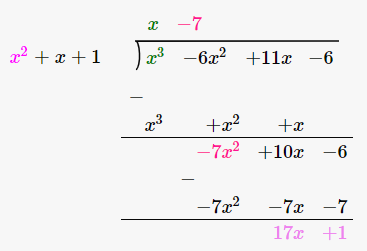Thus,

q(x) = x – 7 and r(x) = 17x -1

(ii) f(x) = 10x4 + 17x3 – 62x2 + 30x – 3, g(x) = 2x2 + 7x + 1

Solution:

Given,

f(x) = 10x4 + 17x3 – 62x2 + 30x – 3 and g(x) = 2x2 + 7x + 1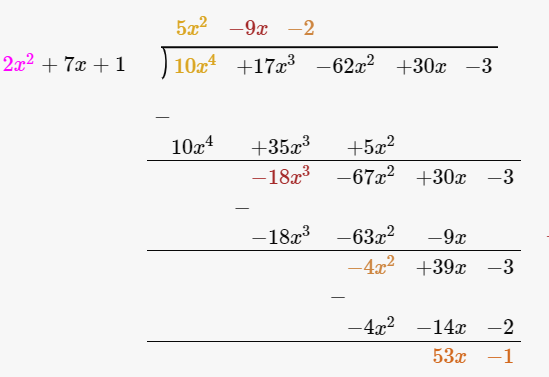Thus,

q(x) = 5x2 – 9x – 2 and r(x) = 53x – 1

(iii) f(x) = 4x3 + 8x2 + 8x + 7, g(x)= 2x2 – x + 1

Solution:

Given,

f(x) = 4x3 + 8x2 + 8x + 7 and g(x)= 2x2 – x + 1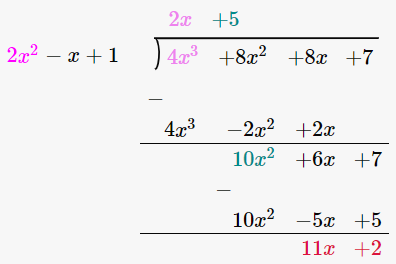Thus,

q(x) = 15x + 10 and r(x) = 3x – 32

(iv) f(x) = 15x3 – 20x2 + 13x – 12, g(x) = x2 – 2x + 2

Solution:

Given,

f(x) = 15x3 – 20x2 + 13x – 12 and g(x) = x2 – 2x + 2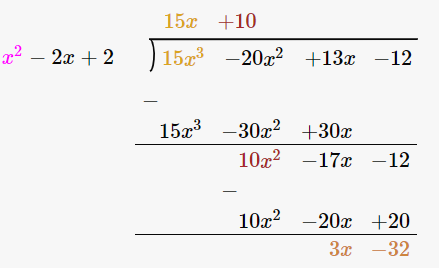Thus,

q(x) = 15x + 10 and r(x) = 3x – 32

2. Check whether the first polynomial is a factor of the second polynomial by applying the division algorithm:

(i) g(t) = t2–3; f(t)=2t4 + 3t3 – 2t2 – 9t – 12

Solution:

Given,

g(t) = t2 – 3; f(t) =2t4 + 3t3 – 2t2 – 9t – 12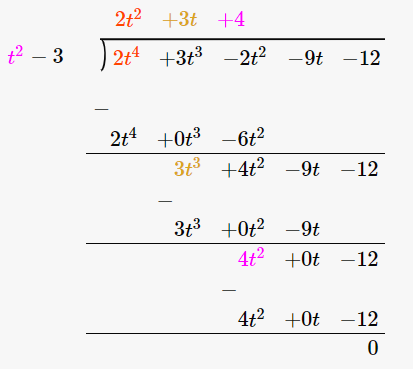Since, the remainder r(t) = 0 we can say that the first polynomial is a factor of the second polynomial.

(ii) g(x) = x3 – 3x + 1; f(x) = x5 – 4x3 + x2 + 3x + 1

Solution:

Given,

g(x) = x3 – 3x + 1; f(x) = x5 – 4x3 + x2 + 3x + 1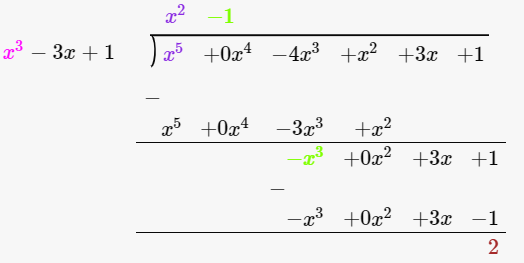Since, the remainder r(x) = 2 and not equal to zero we can say that the first polynomial is not a factor of the second polynomial.

(iii) g(x) = 2x2– x + 3; f(x) = 6x5 − x4 + 4x3 – 5x2 – x –15

Solution:

Given,

g(x) = 2x2– x + 3; f(x)=6x5 − x4 + 4x3 – 5x2 – x –15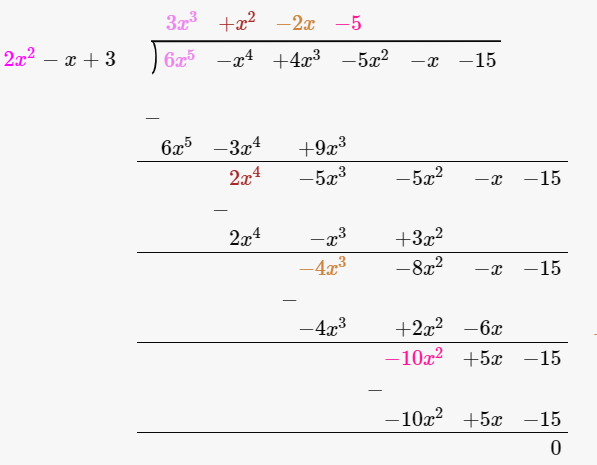Since, the remainder r(x) = 0 we can say that the first polynomial is not a factor of the second polynomial.

3. Obtain all zeroes of the polynomial f(x)= 2x4 + x3 – 14x2 – 19x–6, if two of its zeroes are -2 and -1.

Solution:

Given,

f(x)= 2x4 + x3 – 14x2 – 19x – 6

If the two zeros of the polynomial are -2 and -1, then its factors are (x + 2) and (x + 1)

⇒ (x+2)(x+1) = x2 + x + 2x + 2 = x2 + 3x +2 …… (i)

This means that (i) is a factor of f(x). So, performing division algorithm we get,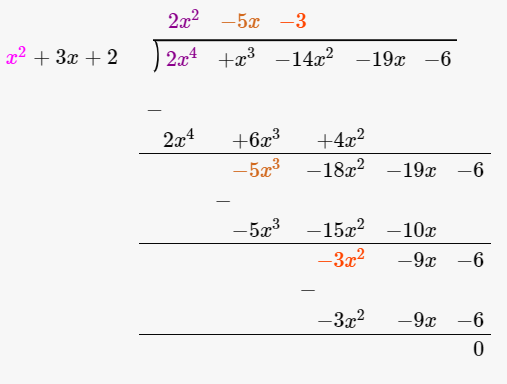The quotient is 2x2 – 5x – 3.

⇒ f(x)= (2x2 – 5x – 3)( x2 + 3x +2)

For obtaining the other 2 zeros of the polynomial

We put,

2x2 – 5x – 3 = 0

⇒ (2x + 1)(x – 3) = 0

∴ x = -1/2 or 3

Hence, all the zeros of the polynomial are -2, -1, -1/2 and 3.

4.  Obtain all zeroes of f(x) = x3 + 13x2 + 32x + 20, if one of its zeros is -2.

Solution:

Given,

f(x)= x3 + 13x2 + 32x + 20

And, -2 is one of the zeros. So, (x + 2) is a factor of f(x),

Performing division algorithm, we get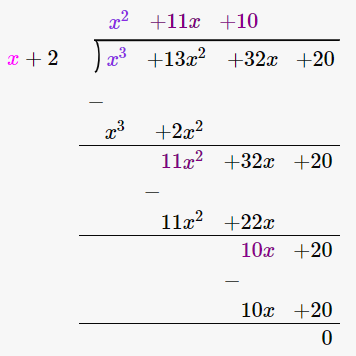⇒ f(x)= (x2 + 11x + 10)( x + 2)

So, putting x2 + 11x + 10 = 0 we can get the other 2 zeros.

⇒ (x + 10)(x + 1) = 0

∴ x = -10 or -1

Hence, all the zeros of the polynomial are -10, -2 and -1.

5. Obtain all zeroes of the polynomial f(x) = x4 – 3x3 – x2 + 9x – 6, if the two of its zeroes are −√3 and √3.

Solution:

Given,

f(x) = x4 – 3x3 – x2 + 9x – 6

Since, two of the zeroes of polynomial are −√3 and √3 so, (x + √3) and (x–√3) are factors of f(x).

⇒ x2 – 3 is a factor of f(x). Hence, performing division algorithm, we get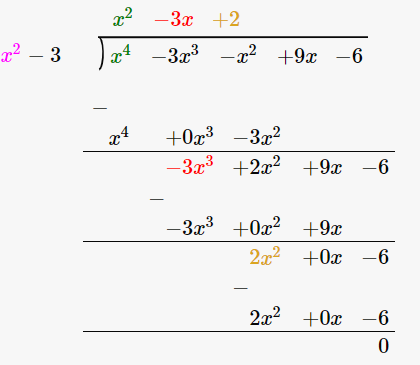⇒ f(x)= (x2 – 3x + 2)( x2 – 3)

So, putting x2 – 3x + 2 = 0 we can get the other 2 zeros.

⇒ (x – 2)(x – 1) = 0

∴ x = 2 or 1

Hence, all the zeros of the polynomial are −√3, 1, √3 and 2.

6.  Obtain all zeroes of the polynomial f(x)= 2x4 – 2x3 – 7x2 + 3x + 6, if the two of its zeroes are −√(3/2) and √(3/2).

Solution:

Given,

f(x)= 2x4 – 2x3 – 7x2 + 3x + 6

Since, two of the zeroes of polynomial are −√(3/2) and √(3/2) so, (x + √(3/2)) and (x –√(3/2)) are factors of f(x).

⇒ x2 – (3/2) is a factor of f(x). Hence, performing division algorithm, we get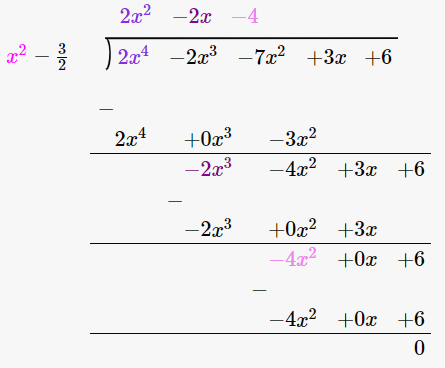⇒ f(x)= (2x2 – 2x – 4)( x2 – 3/2)= 2(x2 – x – 2)( x2 – 3/2)

So, putting x2 – x – 2 = 0 we can get the other 2 zeros.

⇒ (x – 2)(x + 1) = 0

∴ x = 2 or -1

Hence, all the zeros of the polynomial are −√(3/2), -1, √(3/2) and 2.

7.  Find all the zeroes of the polynomial x4 + x3 – 34x2 – 4x + 120, if the two of its zeros are 2 and -2.

Solution:

Let,

f(x) = x4 + x3 – 34x2 – 4x + 120

Since, two of the zeroes of polynomial are −2 and 2 so, (x + 2) and (x – 2) are factors of f(x).

⇒ x2 – 4 is a factor of f(x). Hence, performing division algorithm, we get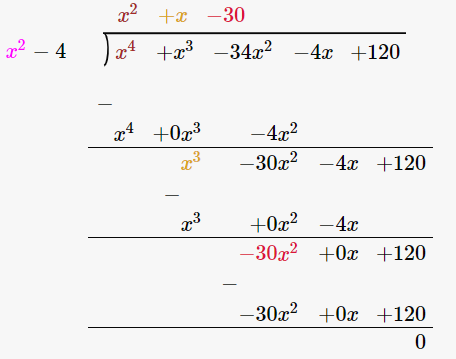⇒ f(x)= (x2 + x – 30)( x2 – 4)

So, putting x2 + x – 30 = 0 we can get the other 2 zeros.

⇒ (x – 6)(x + 5) = 0

∴ x = 6 or -5

Hence, all the zeros of the polynomial are -5, -2, 2 and 6.